# NCERT Exemplar Class 8 Maths Solutions for Chapter 3 - Squares And Square Roots Cubes And Cube Roots

## NCERT Exemplar Solutions Class 8 Maths Chapter 3 – Free PDF Download

NCERT Exemplar Class 8 Maths Chapter 3 Squares and Square Roots & Cubes and Cube Roots, is provided here for students to prepare for exams. These exemplars problems and solutions are designed by experts in accordance with CBSE syllabus(2021-2022) for 8th standard, which covers all the topics of Maths Chapter 3. In this chapter, the students will learn about what are square roots and cube roots of a number and how to find the square roots and cube roots of a given number. To master the concepts of square roots and cube roots students must solve the questions from exemplars for Maths class 8. Solving the Exemplar questions for Class 8 will help the students to understand the concepts present in each chapter. Below is the list of topics covered in Chapter 3 of Class 8, Maths subject.

• Squares and square roots
• Properties of square numbers
• Find the squares of a number
• Pythagorean triplets
• Finding the square root of a number in general form by prime factorization and by division method
• Cubes and their prime factors
• Finding a perfect cube
• Finding cube roots by prime factorization method and estimation method

Solve these exemplars and score good marks in the main exams. Also, it is suggested to solve sample papers and previous year question papers which gives an idea about types of questions asked in the board exam from Squares and Square Roots & Cubes and Cube Roots. BYJU’S also provide notes, exemplar books, Maths NCERT Solutions for 8th standard and question papers to help students practice well.

### NCERT Exemplar Class 8 Maths Solutions Chapter 3 Squares And Square Roots Cubes And Cube Roots:-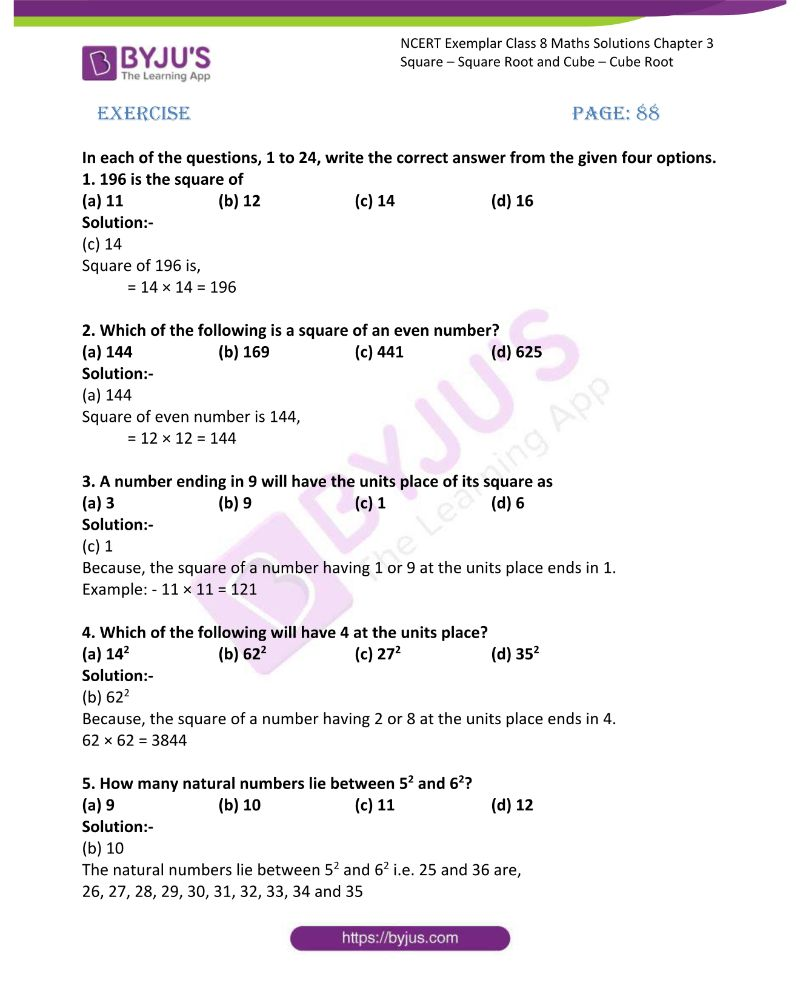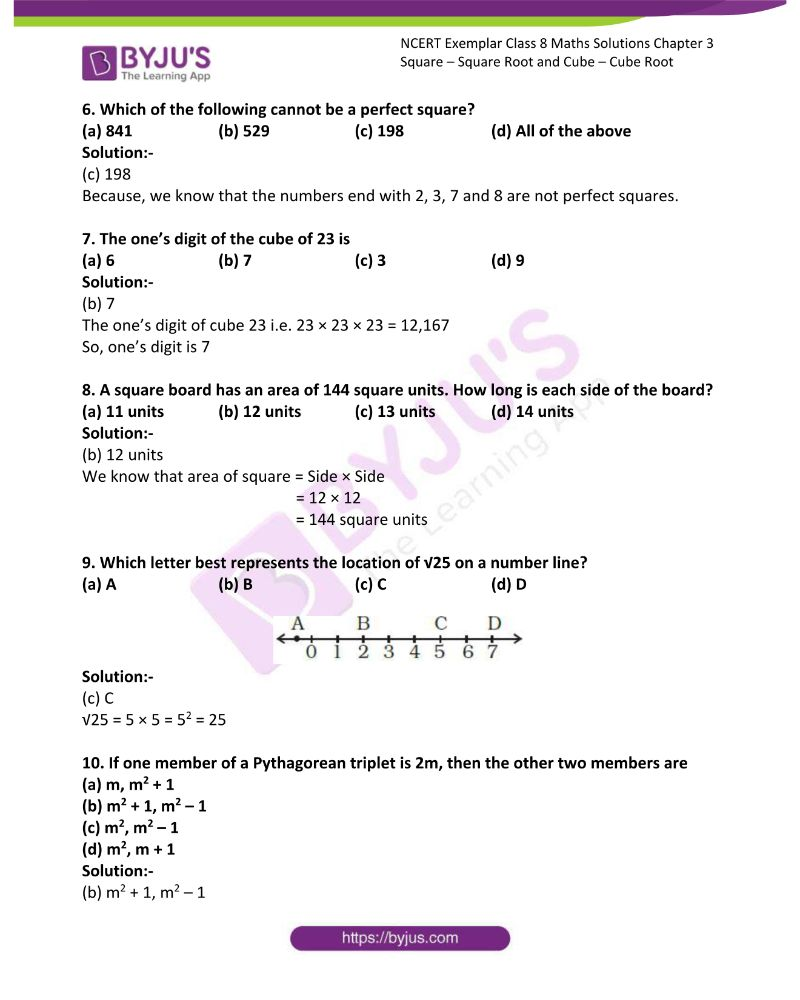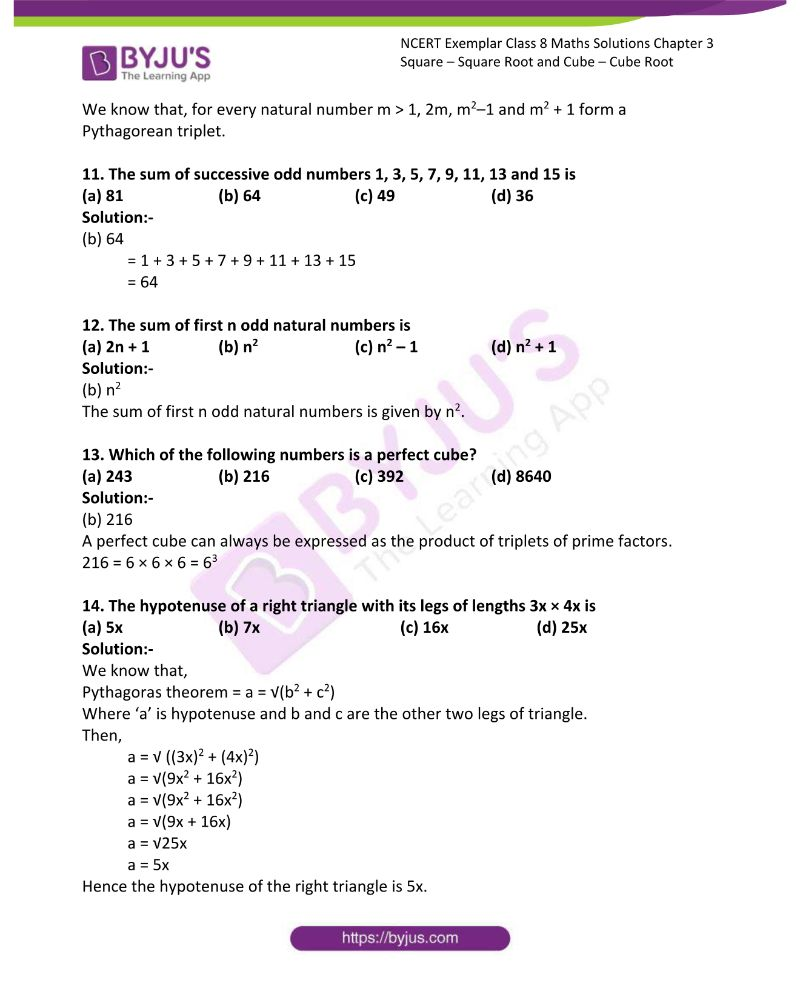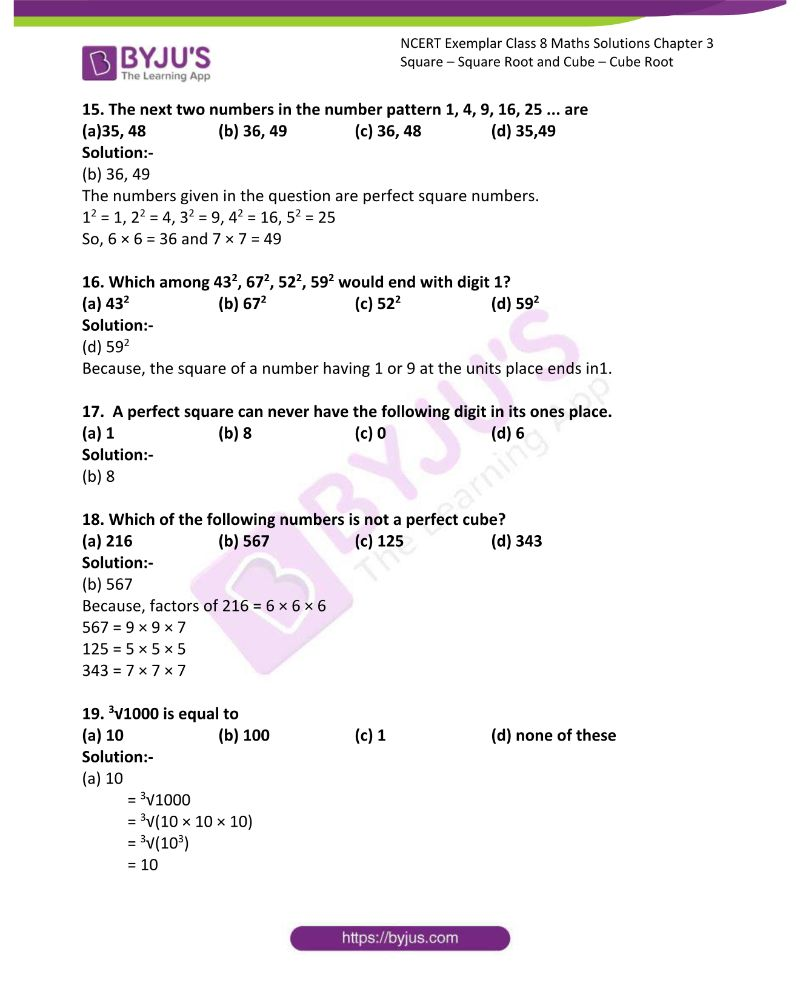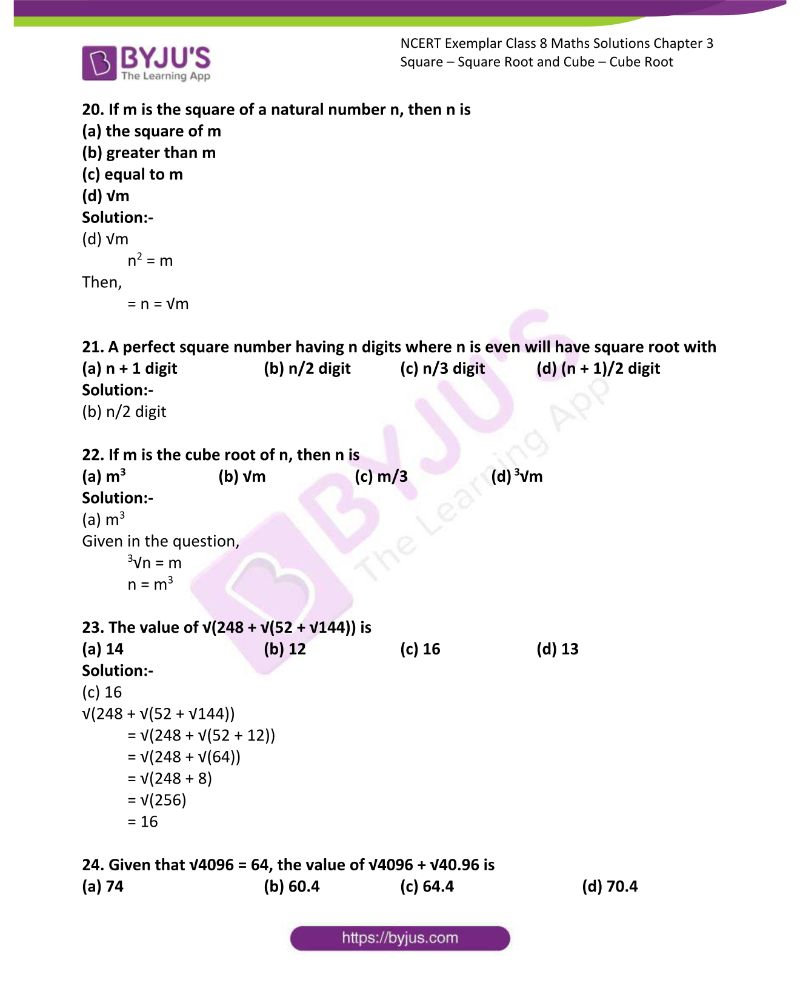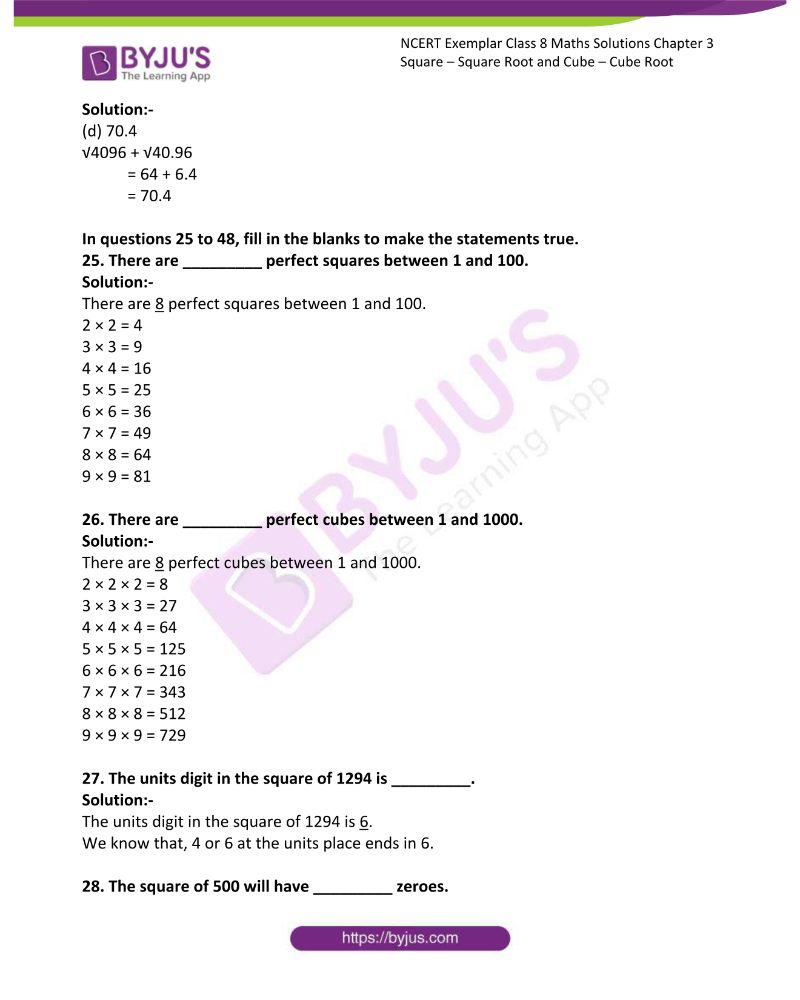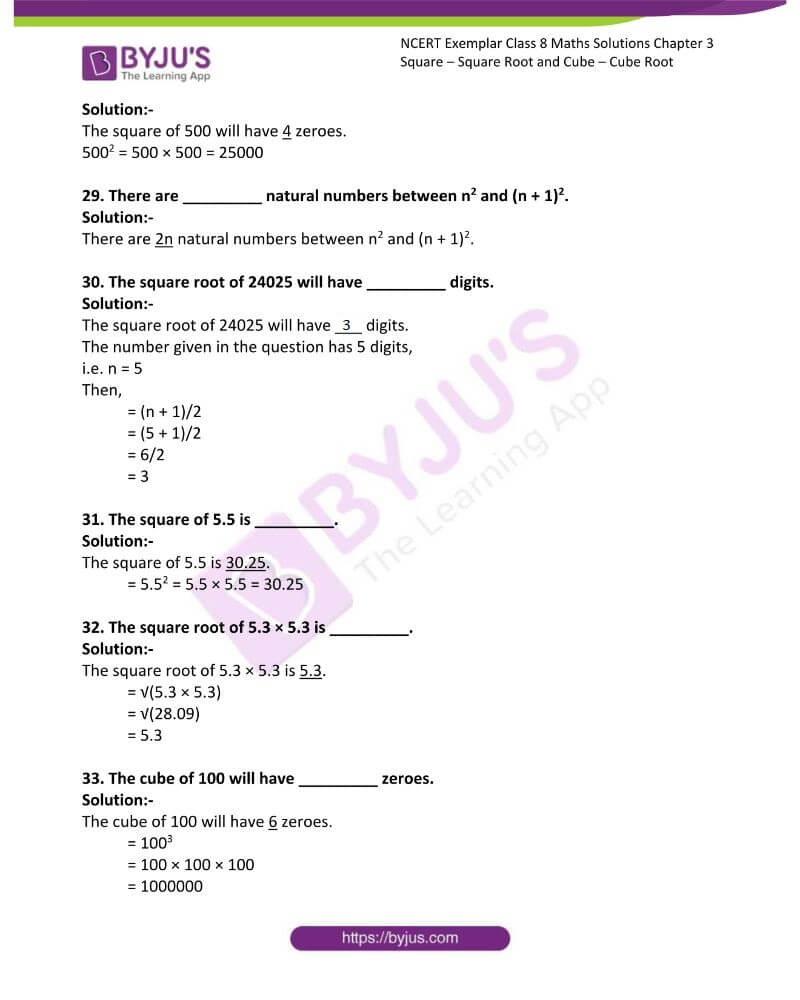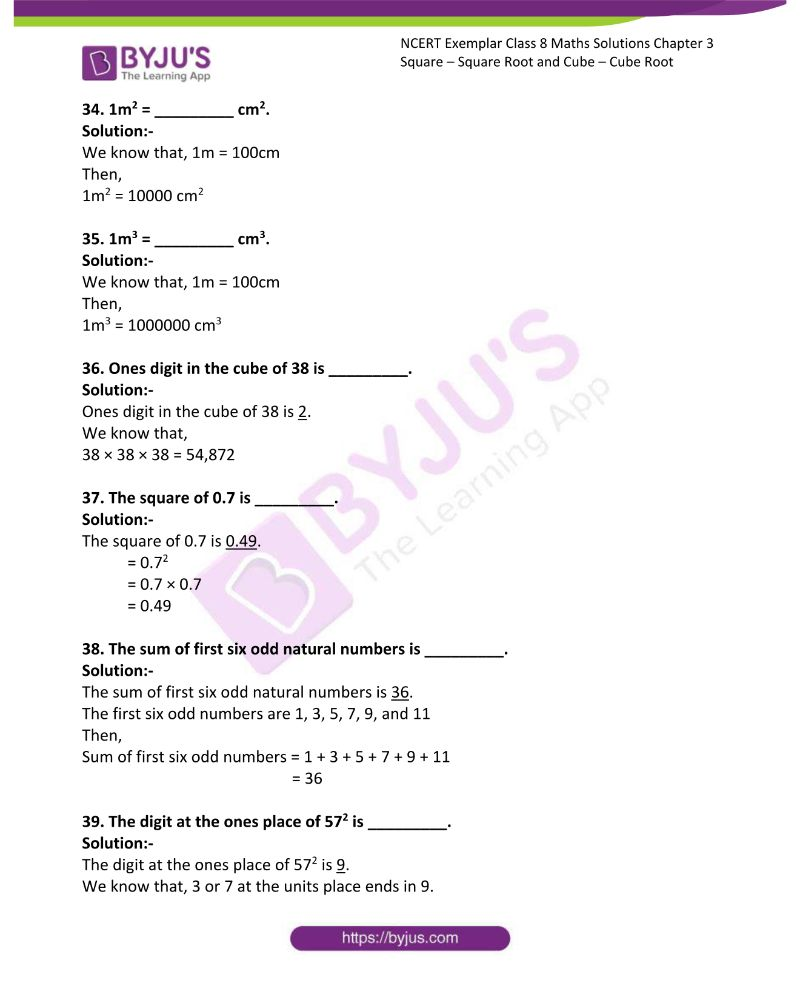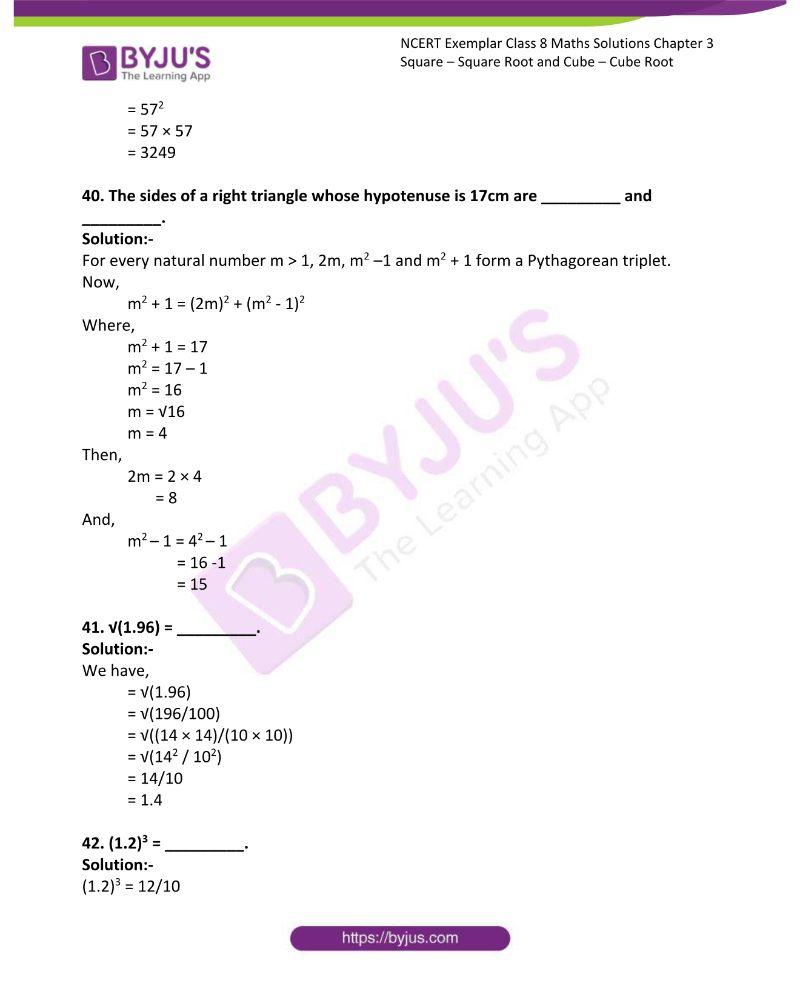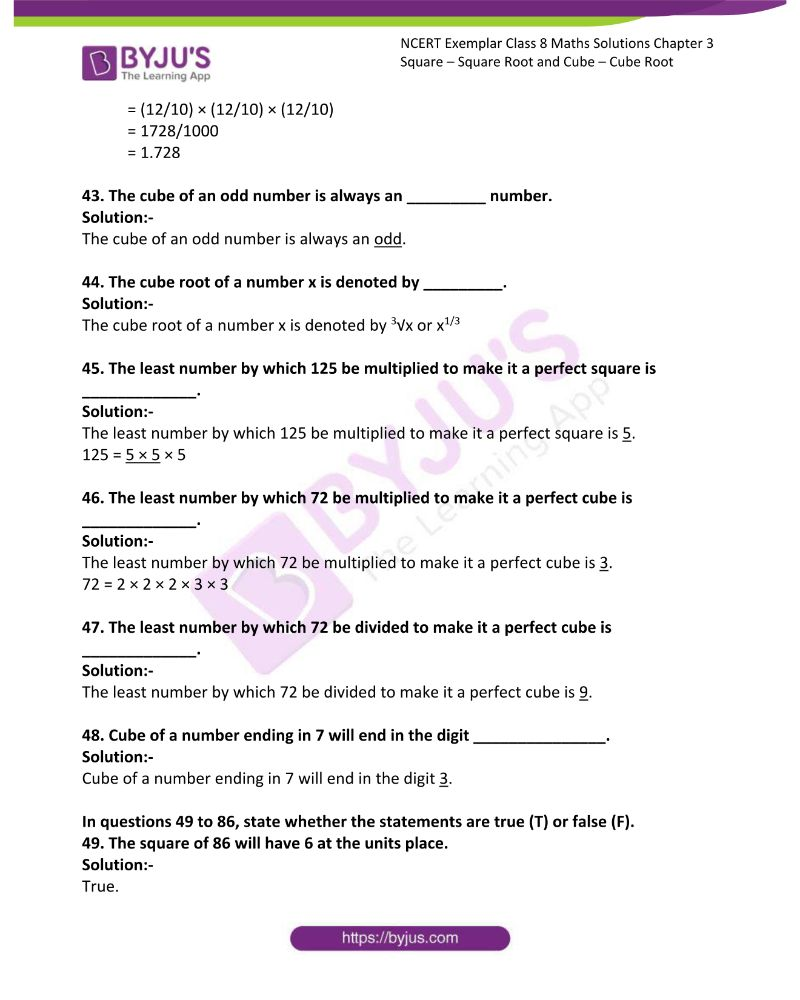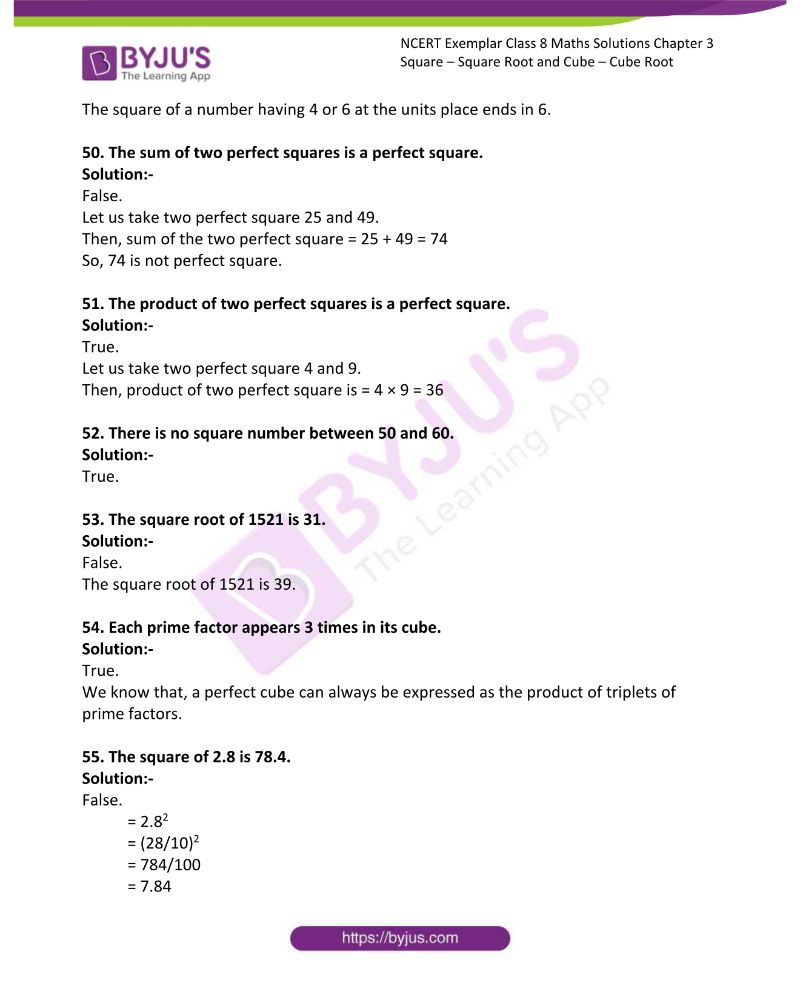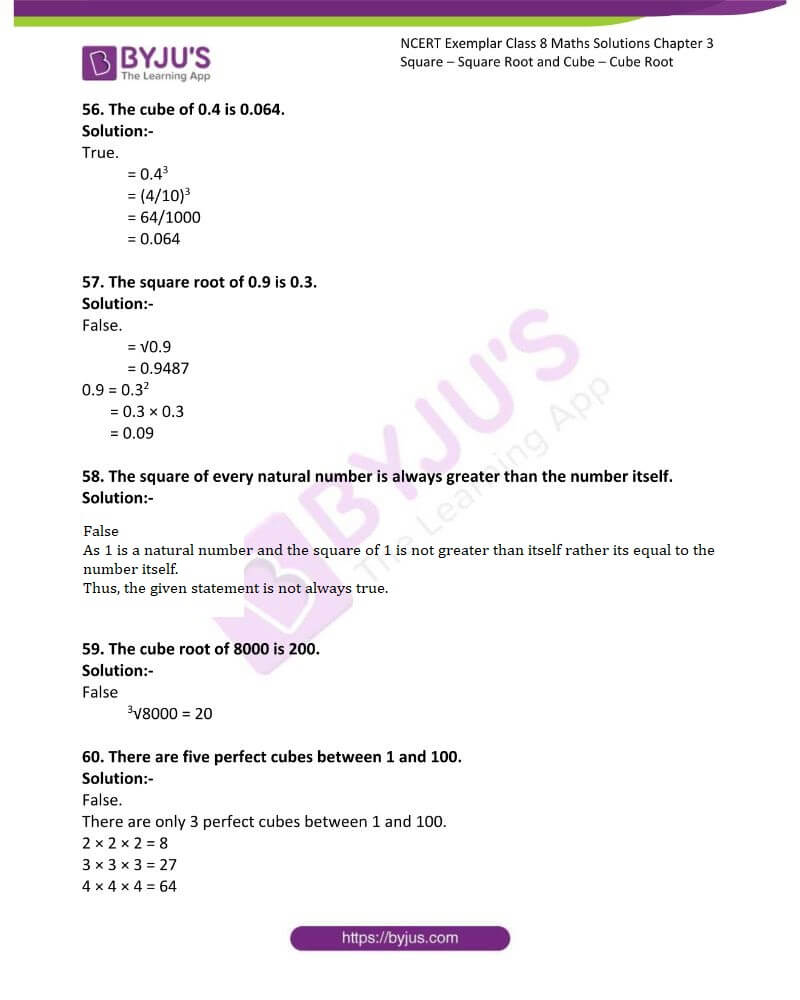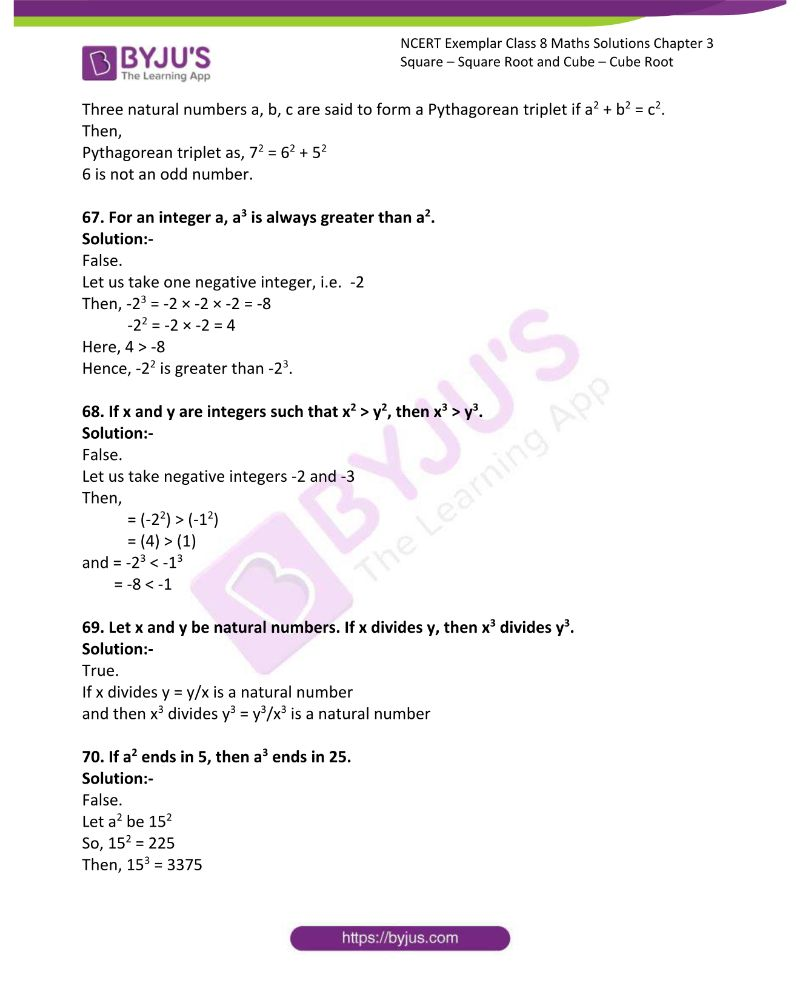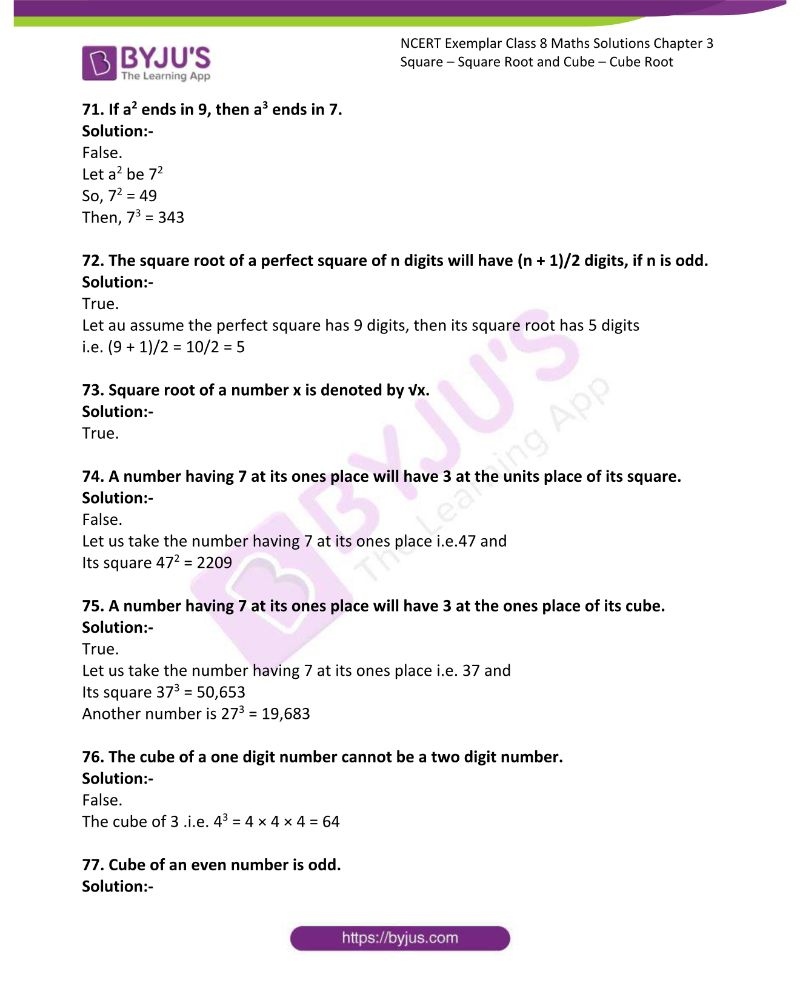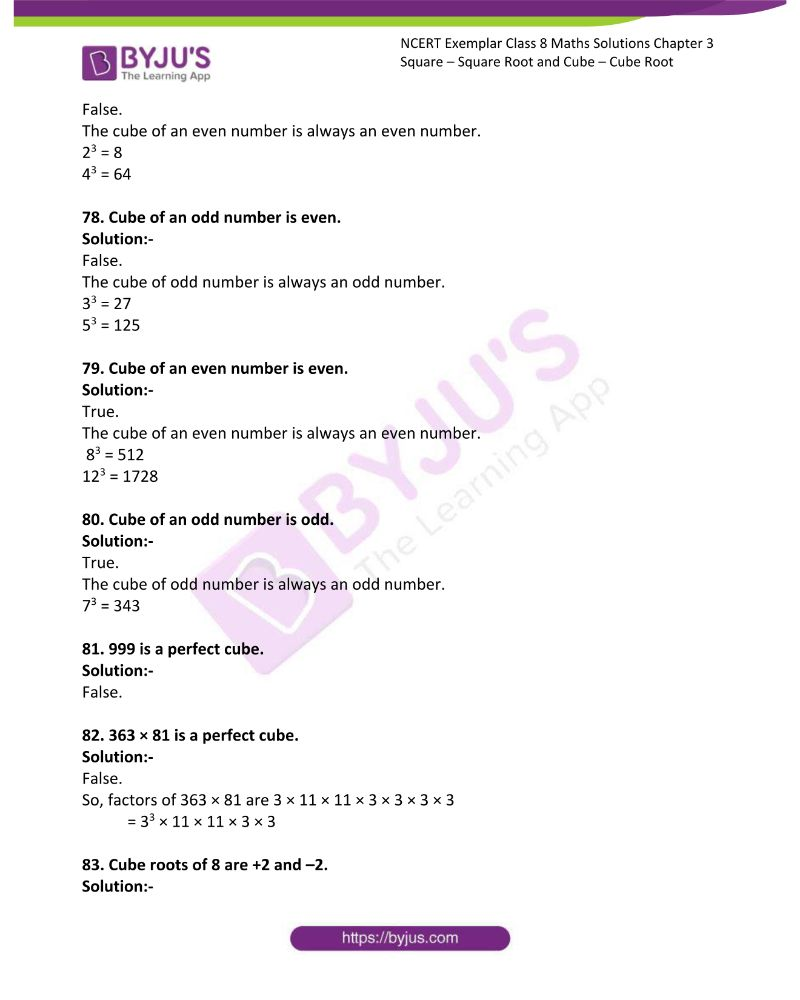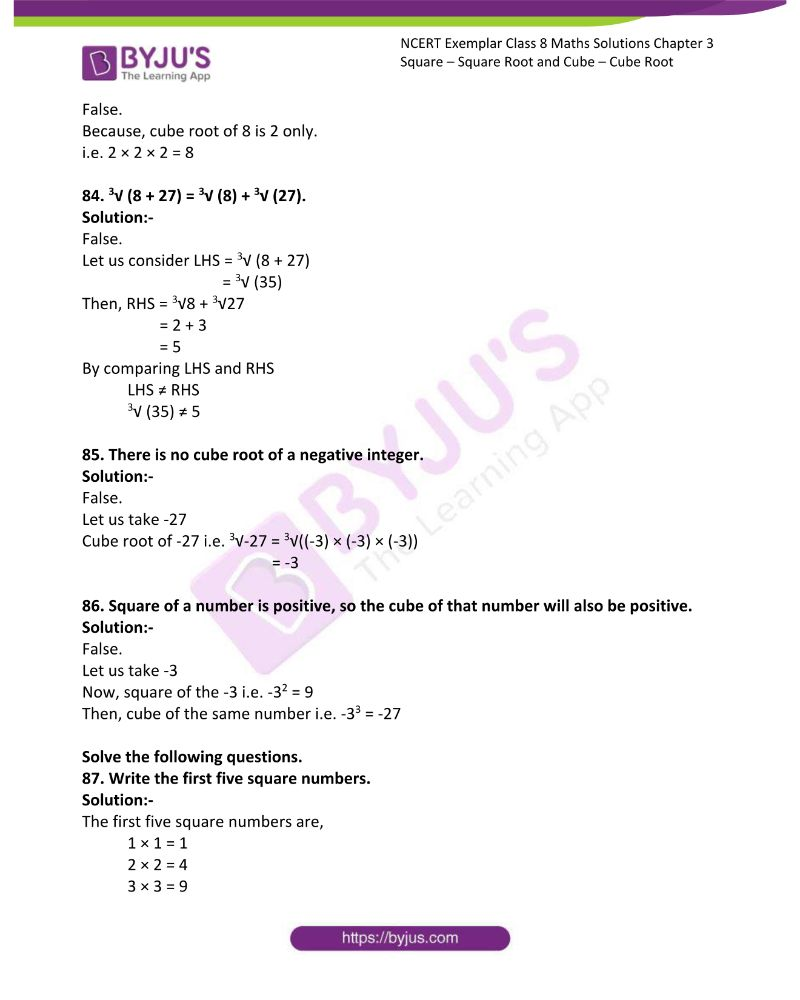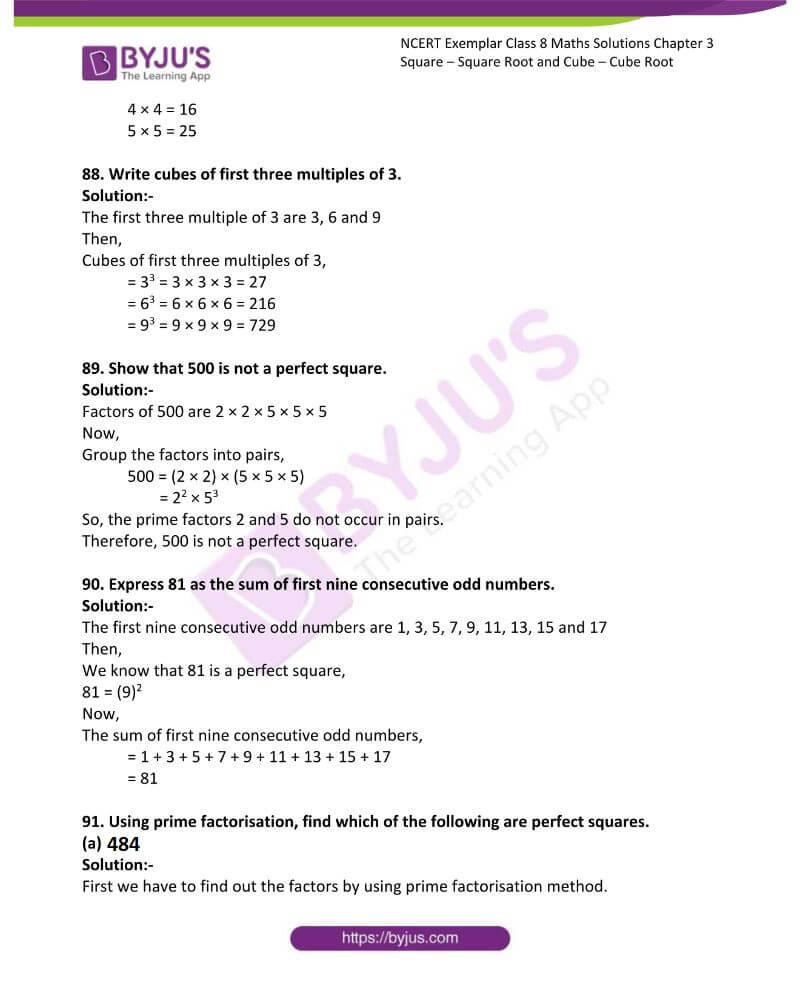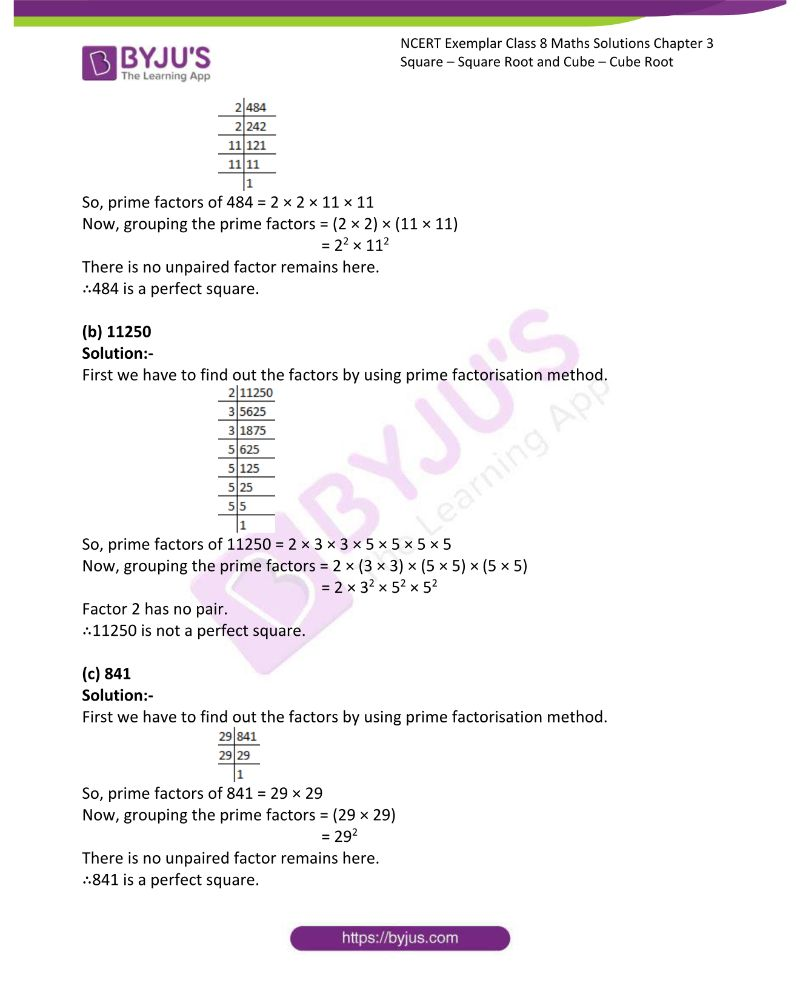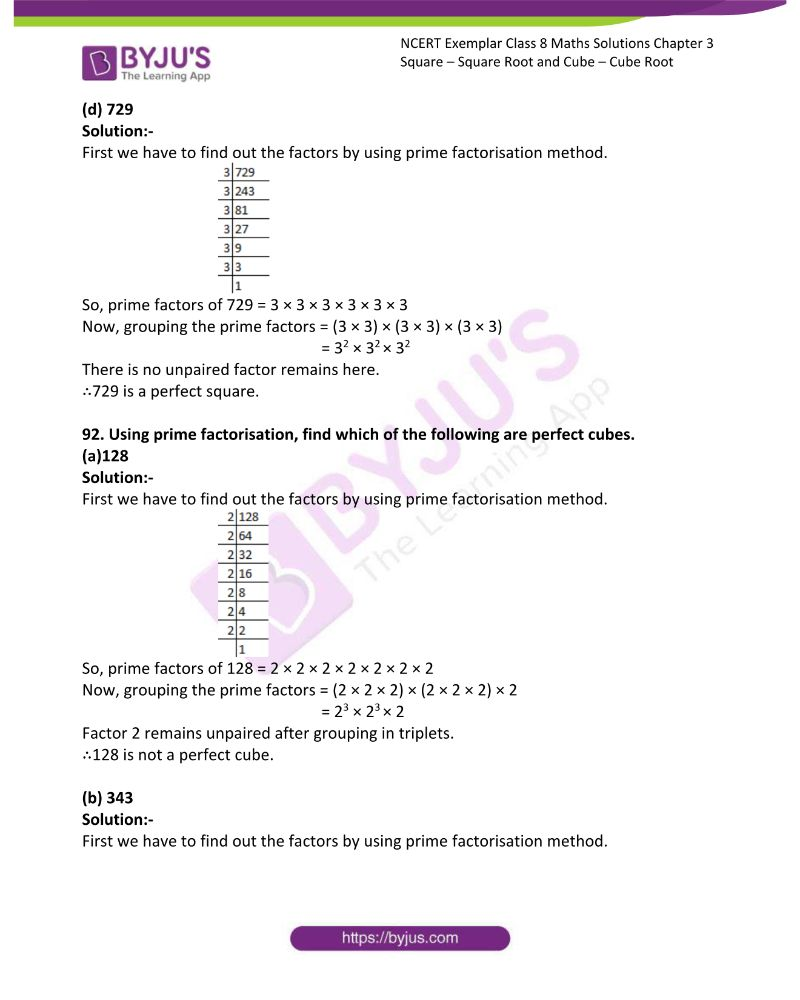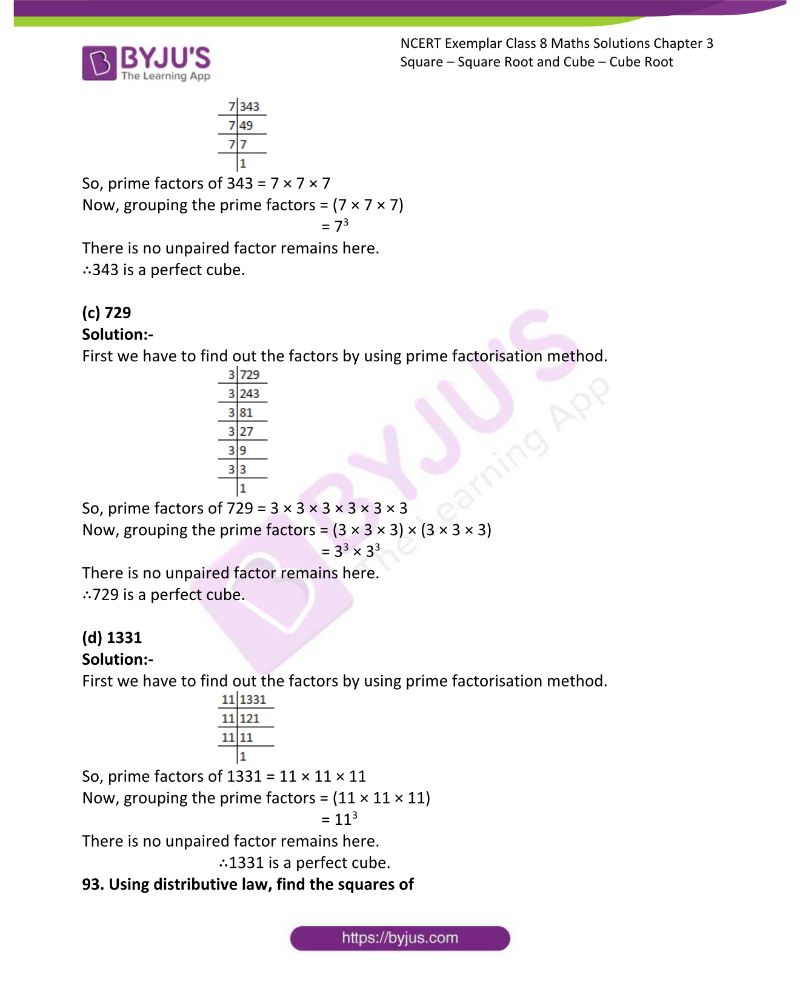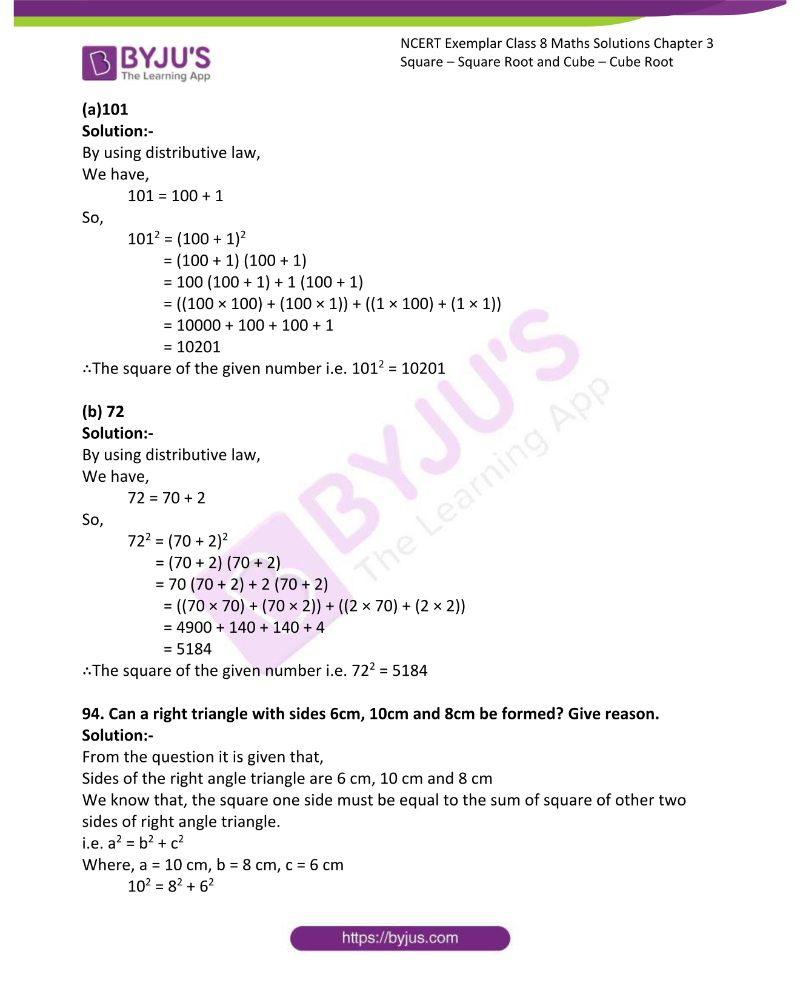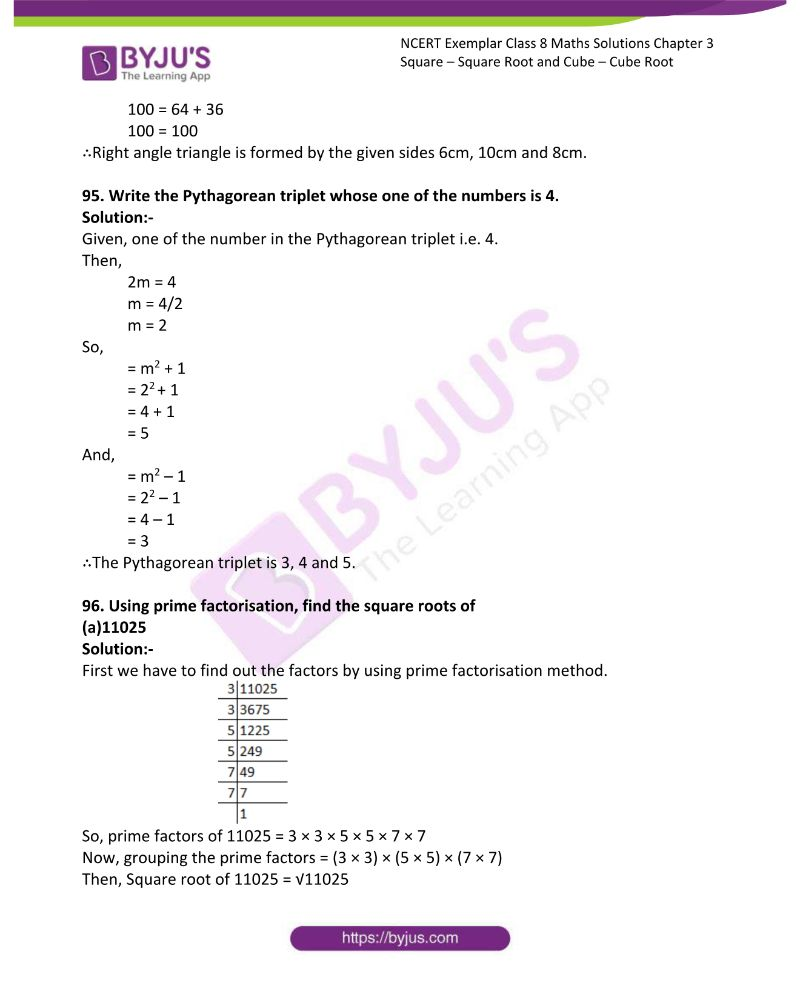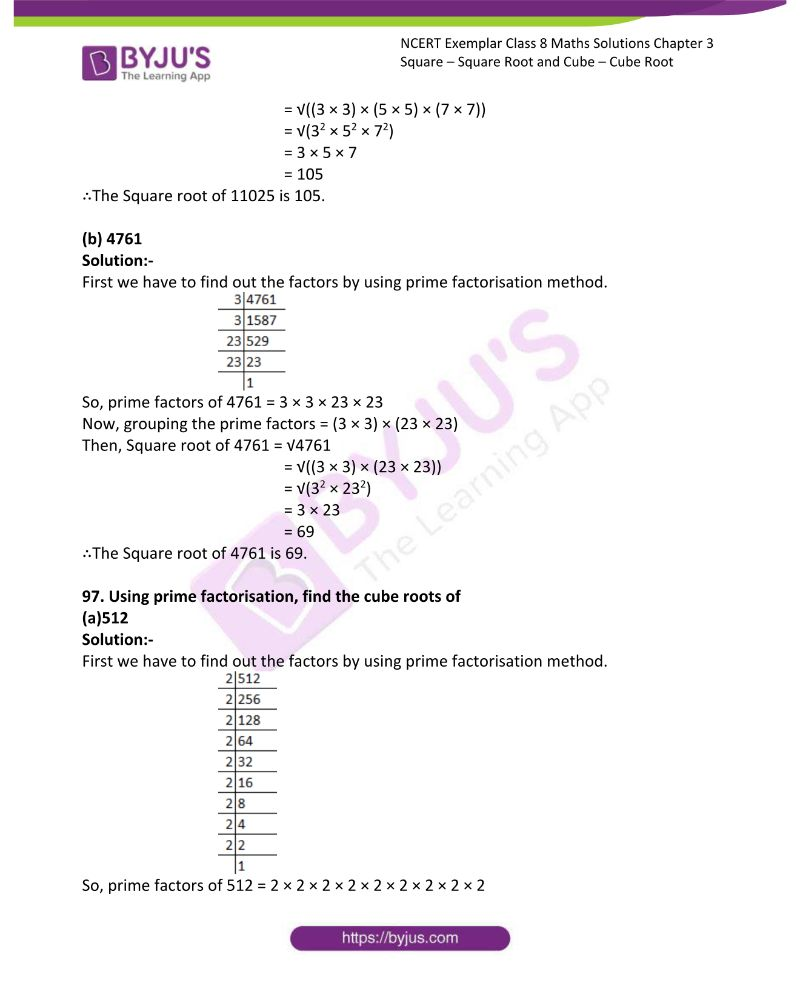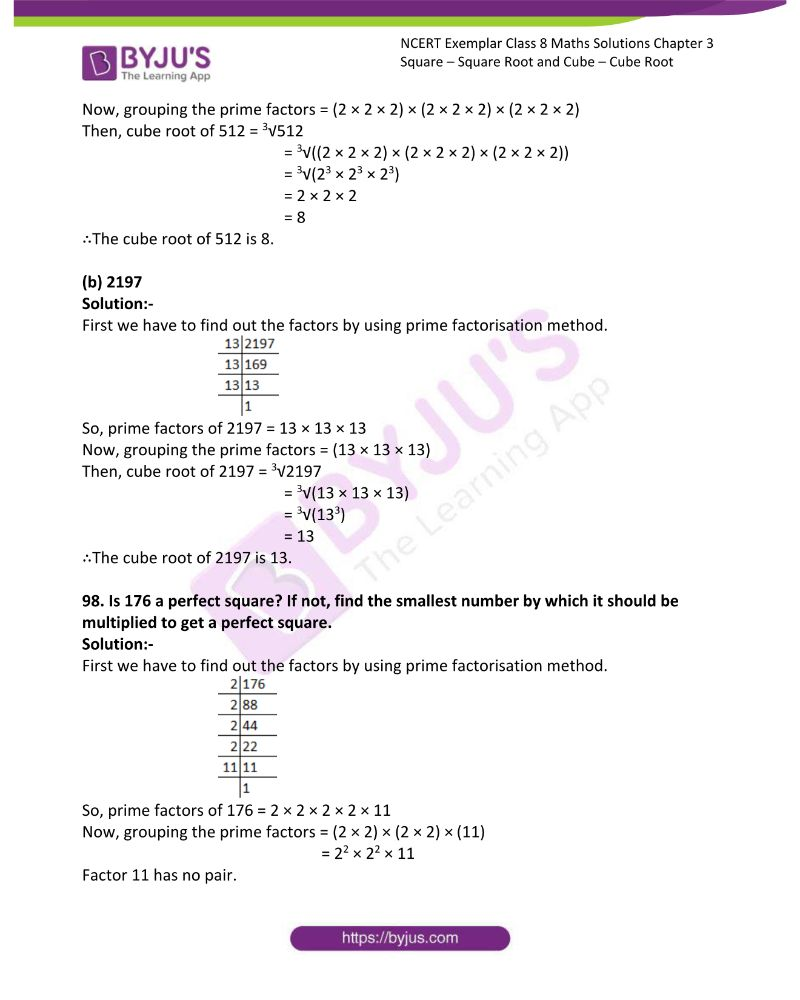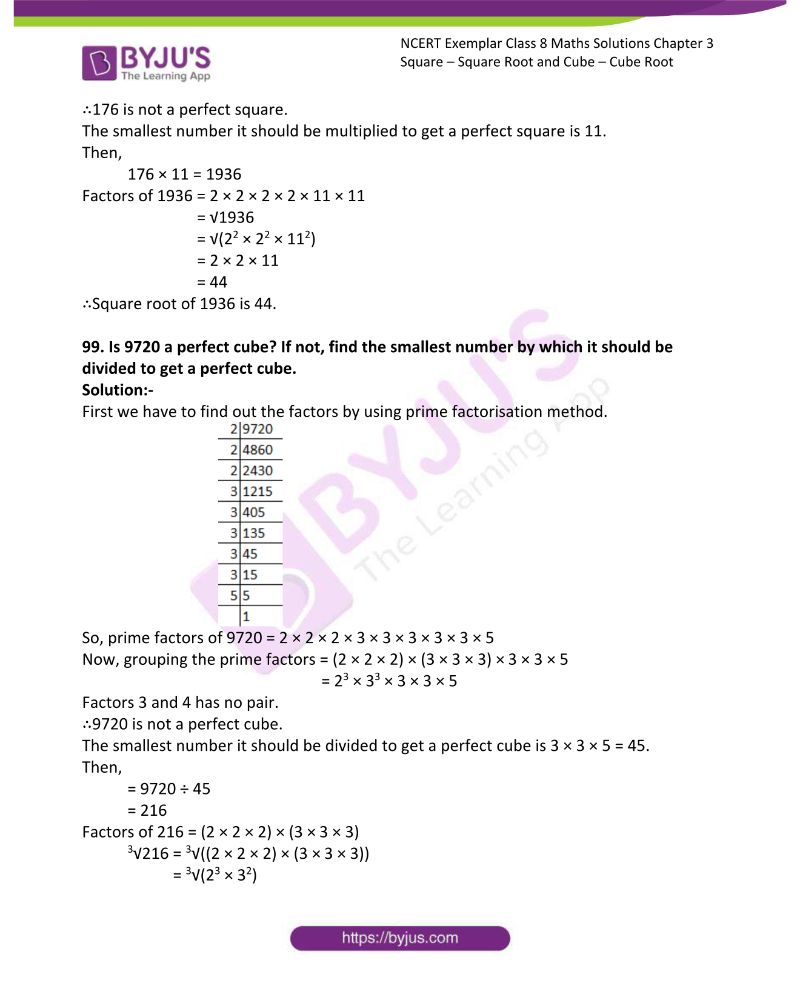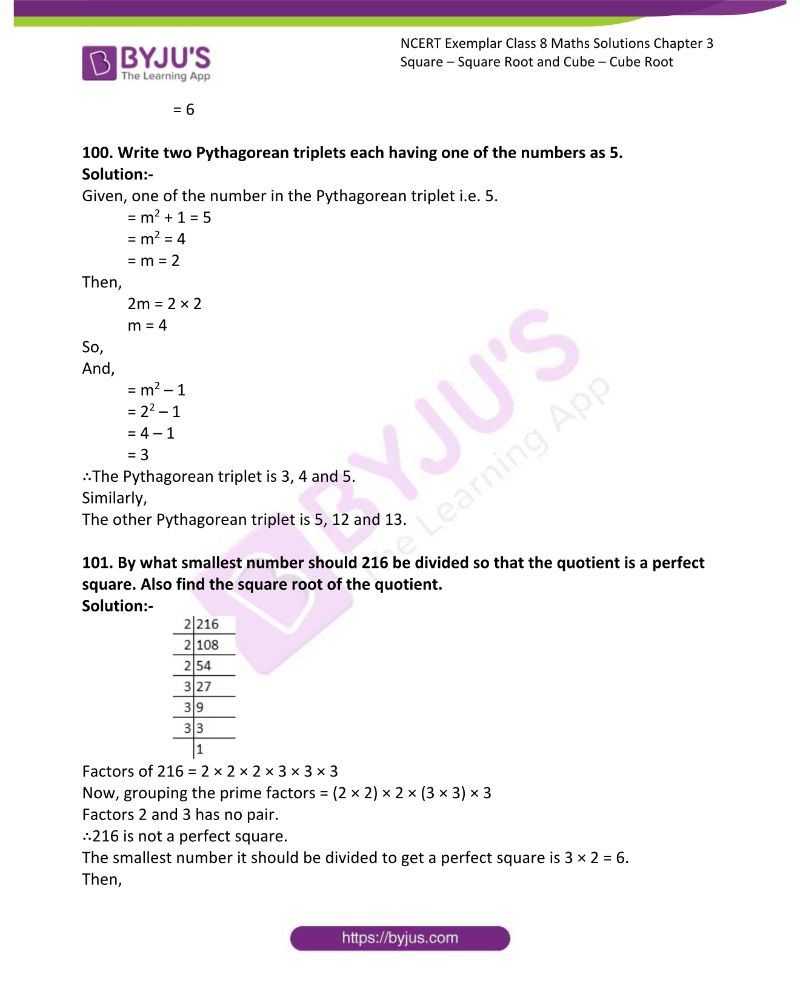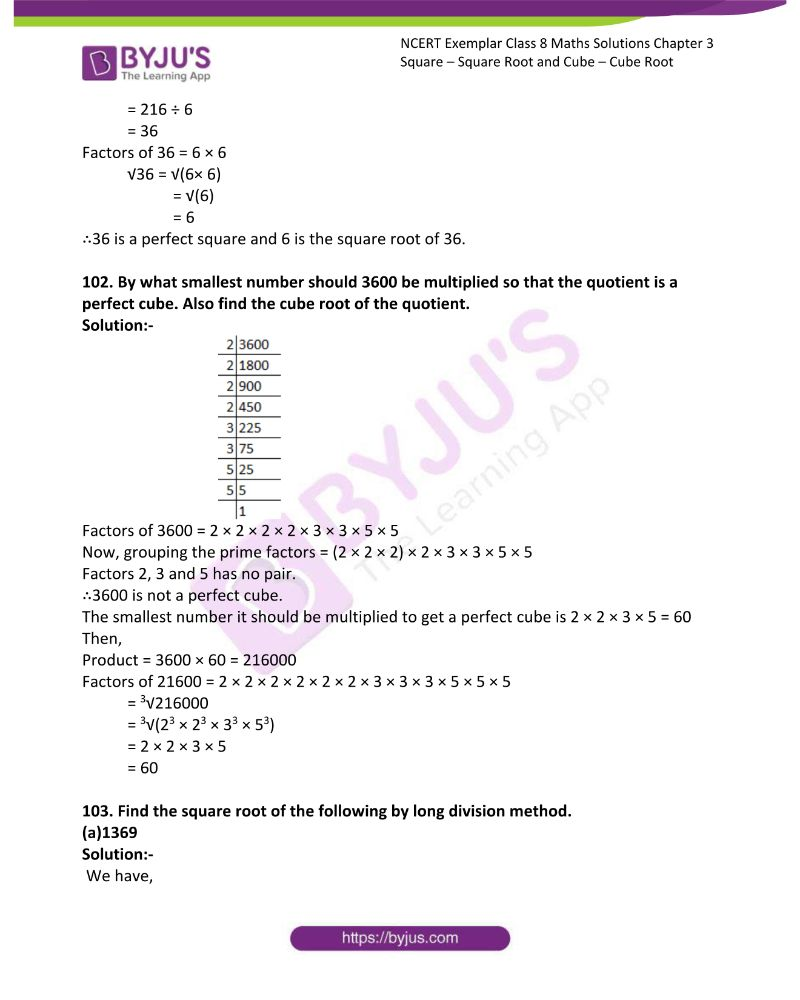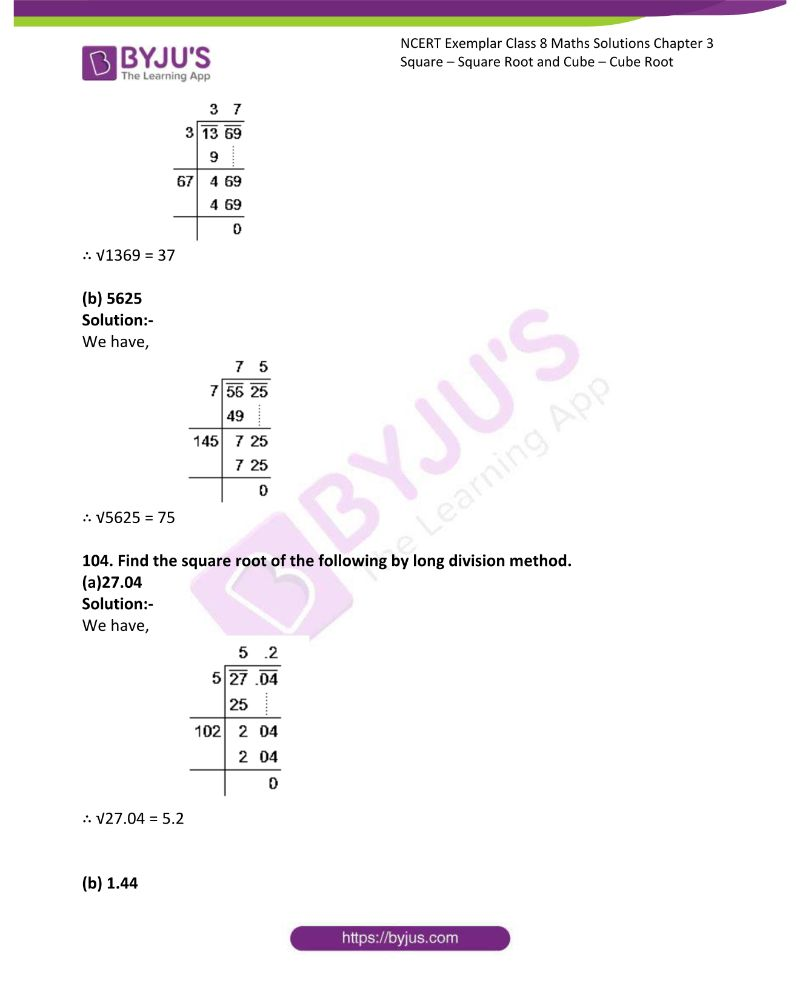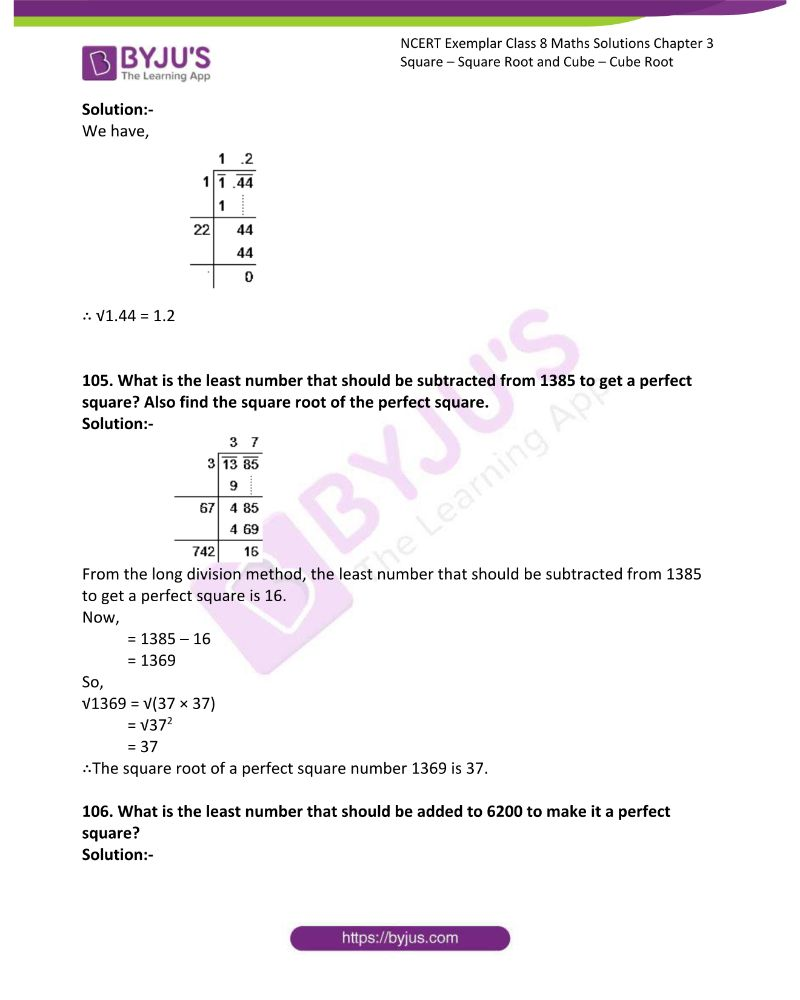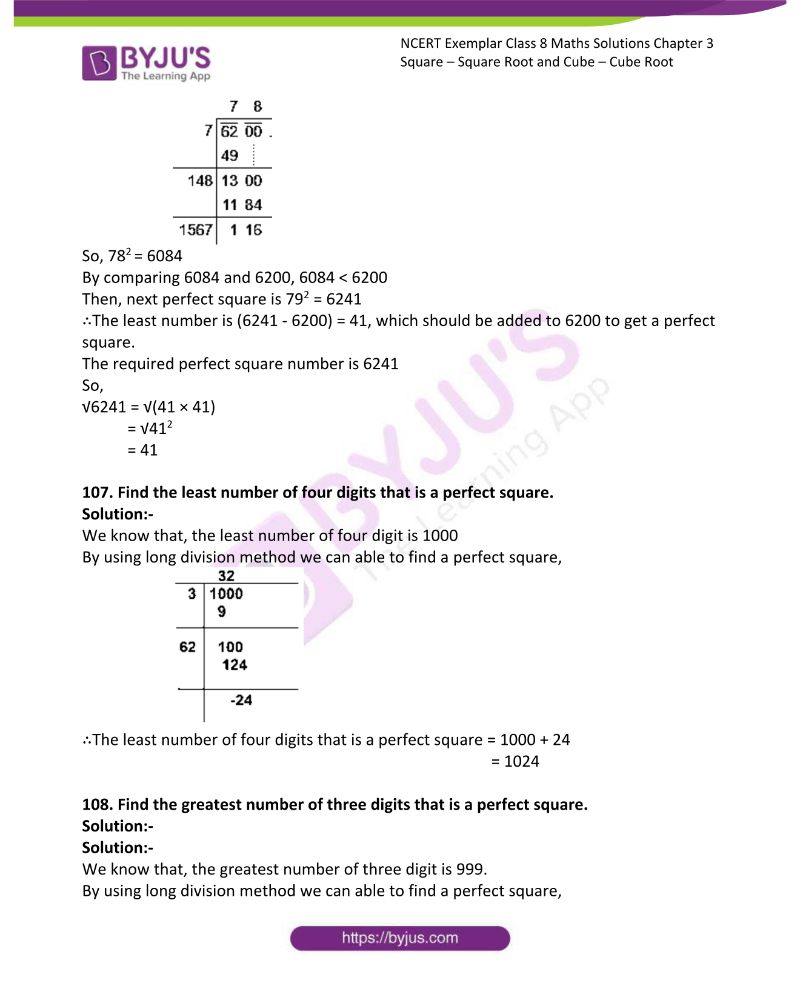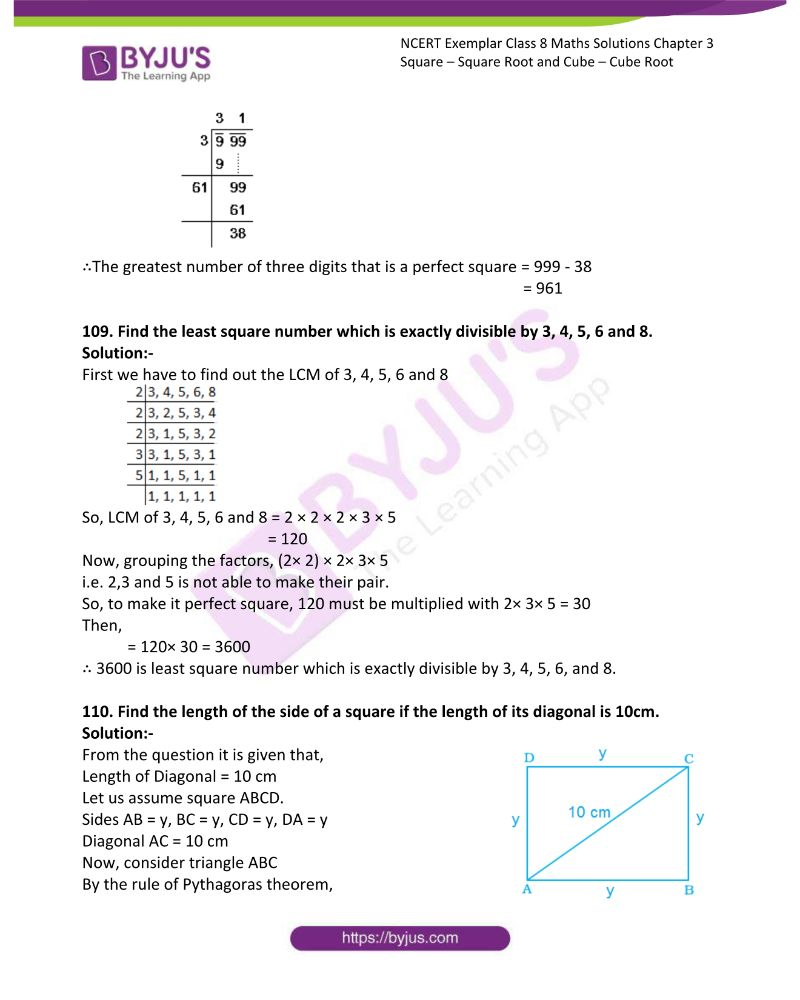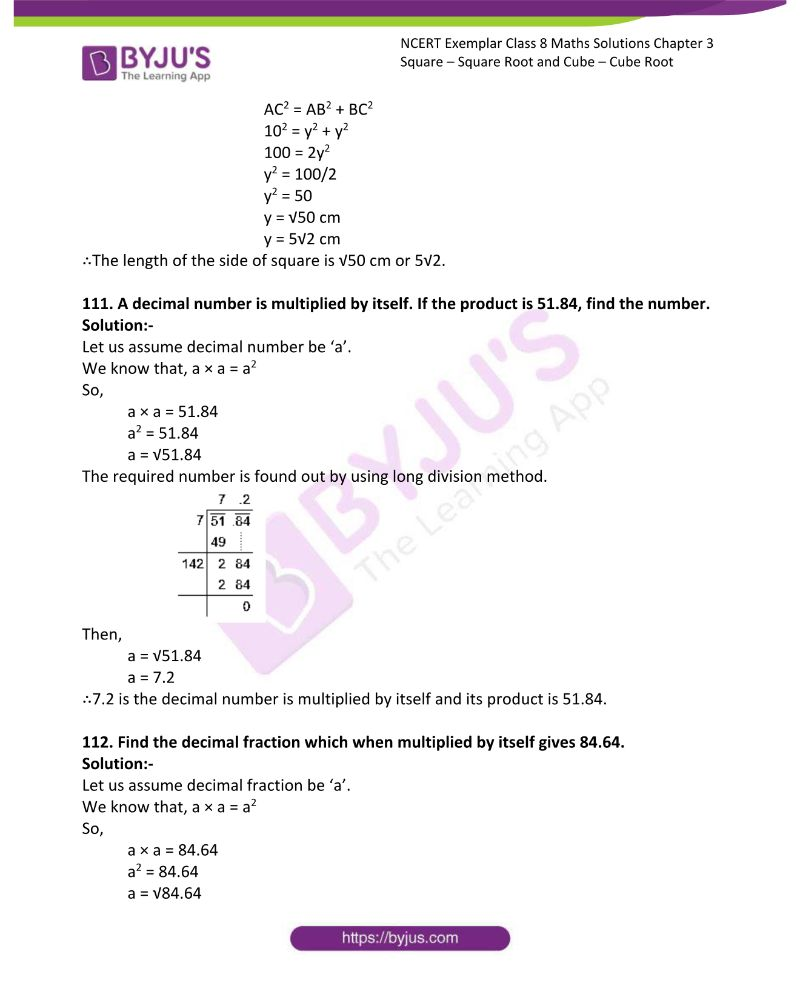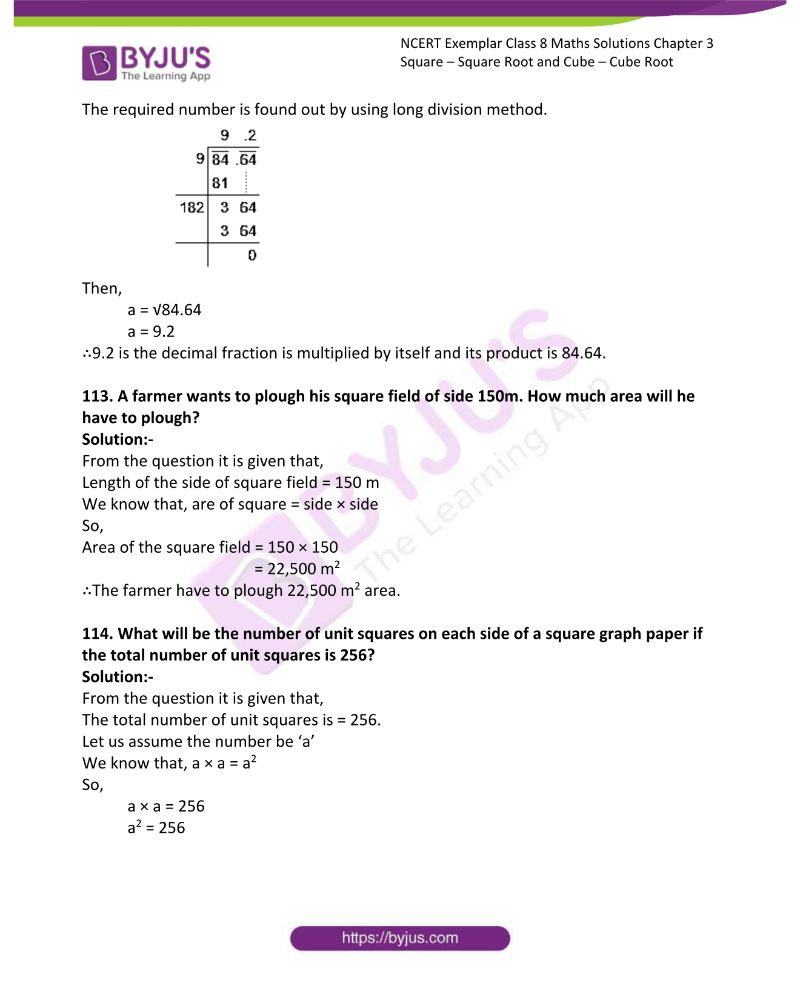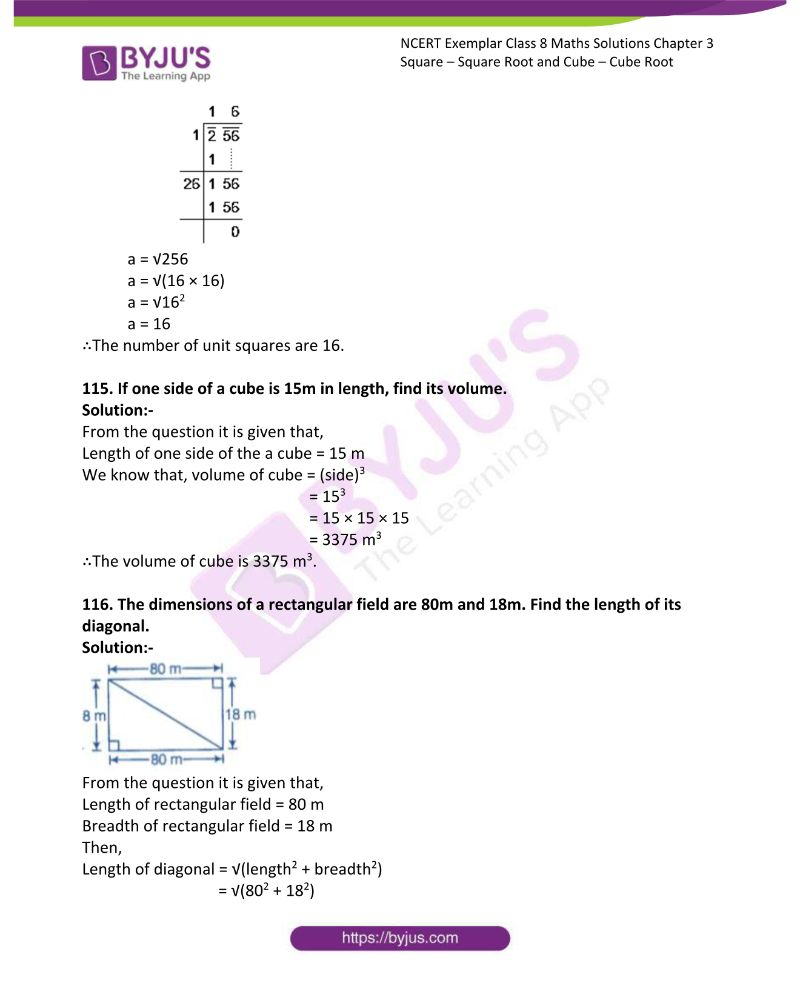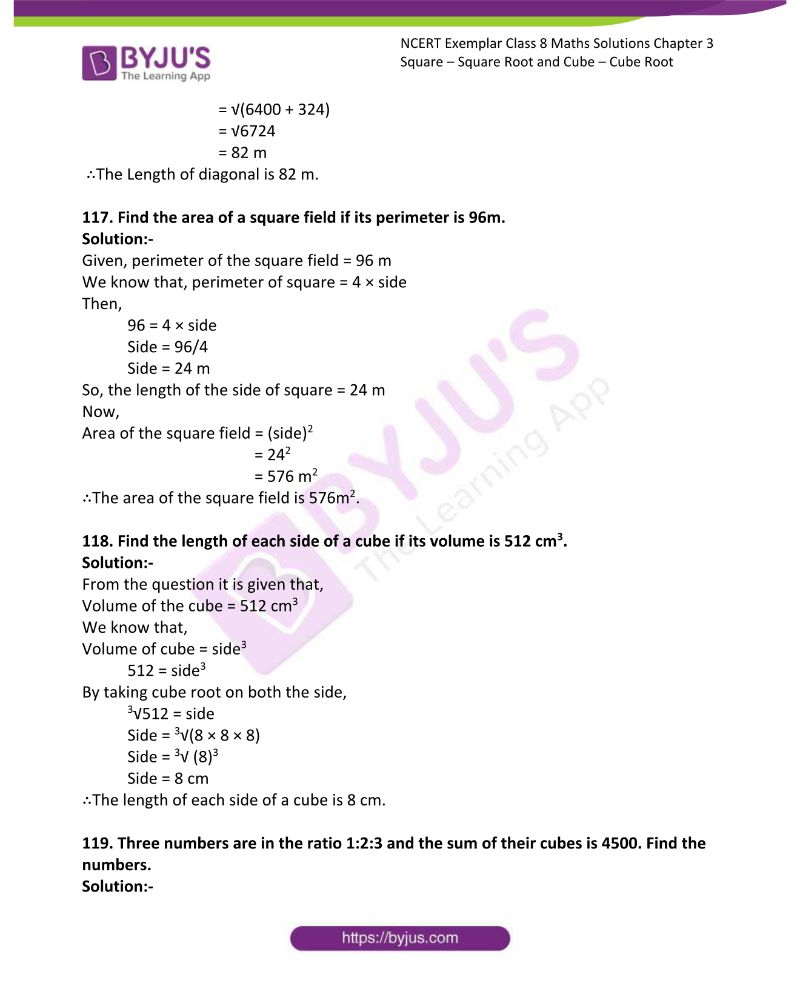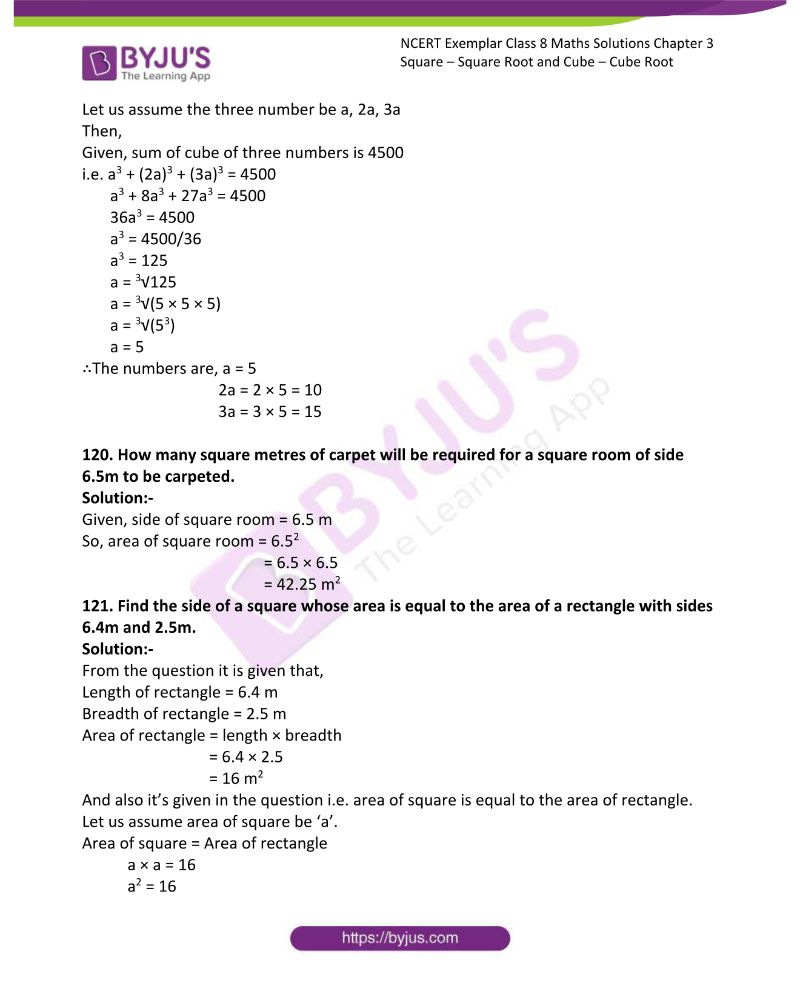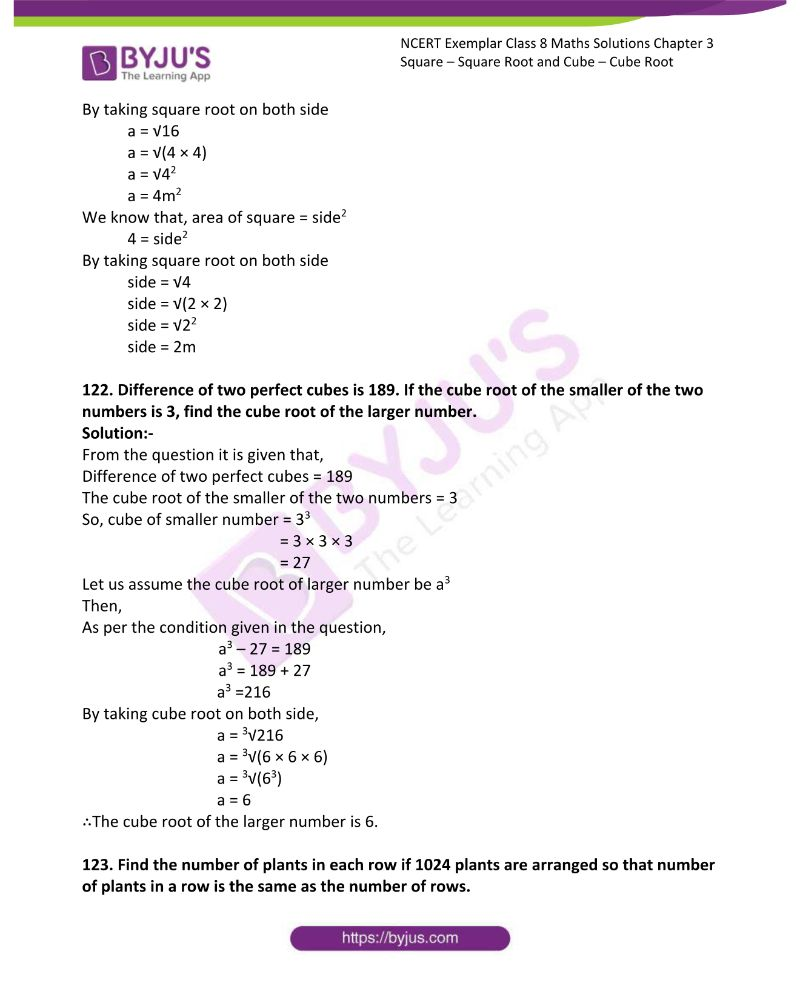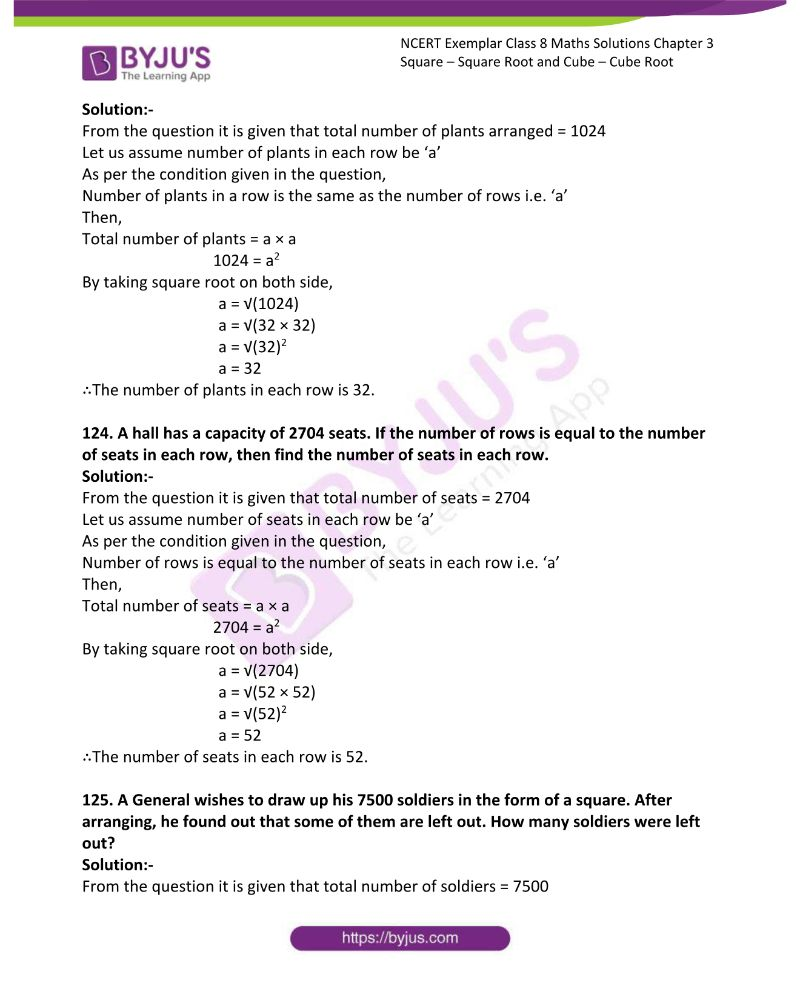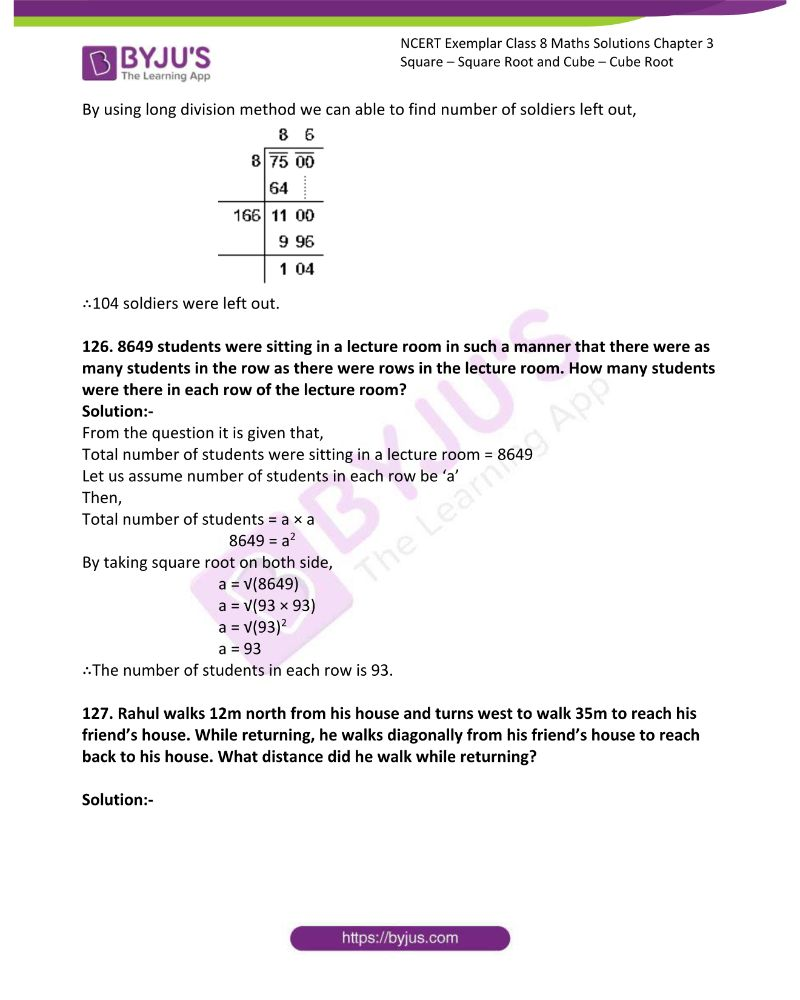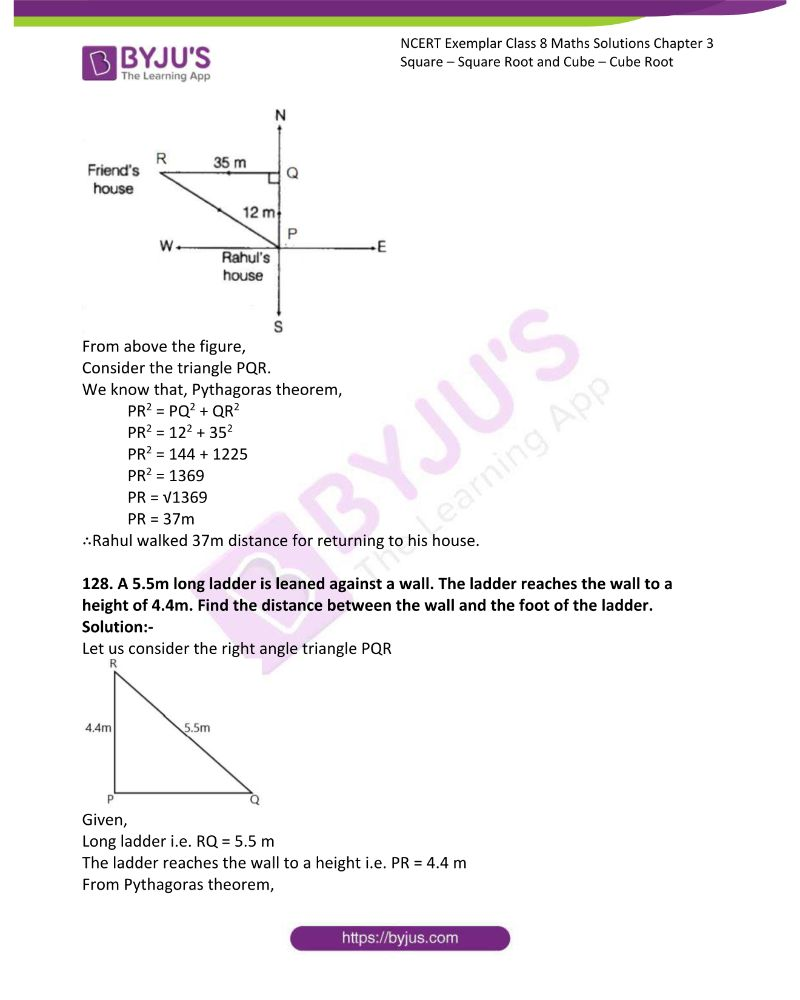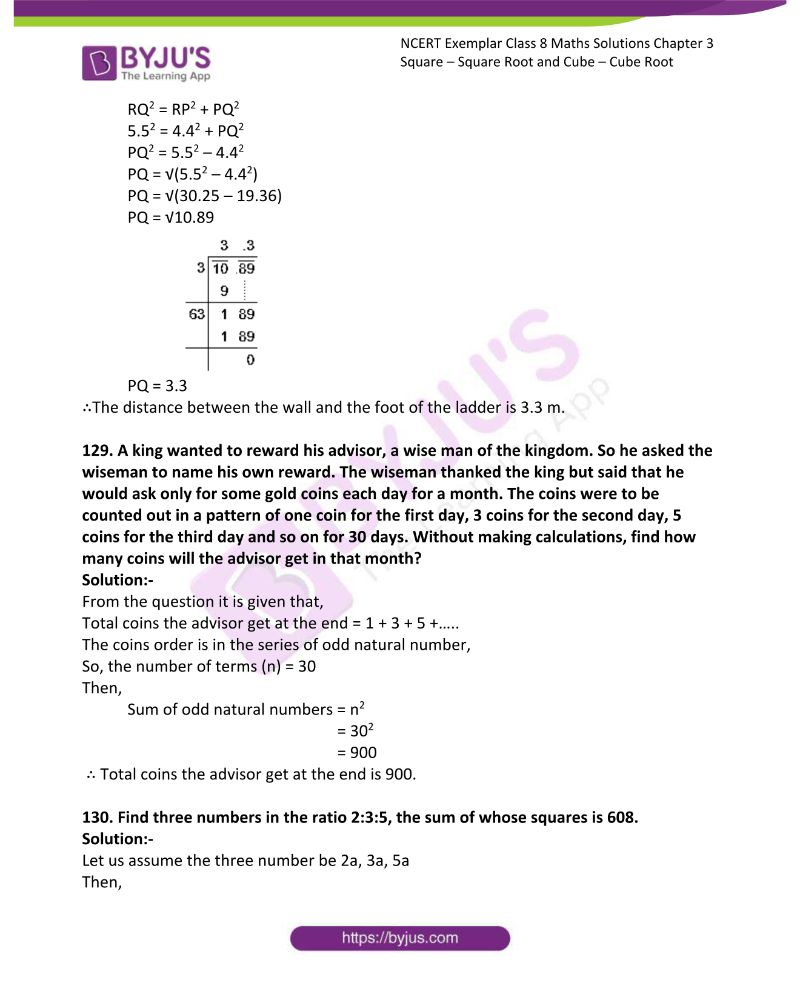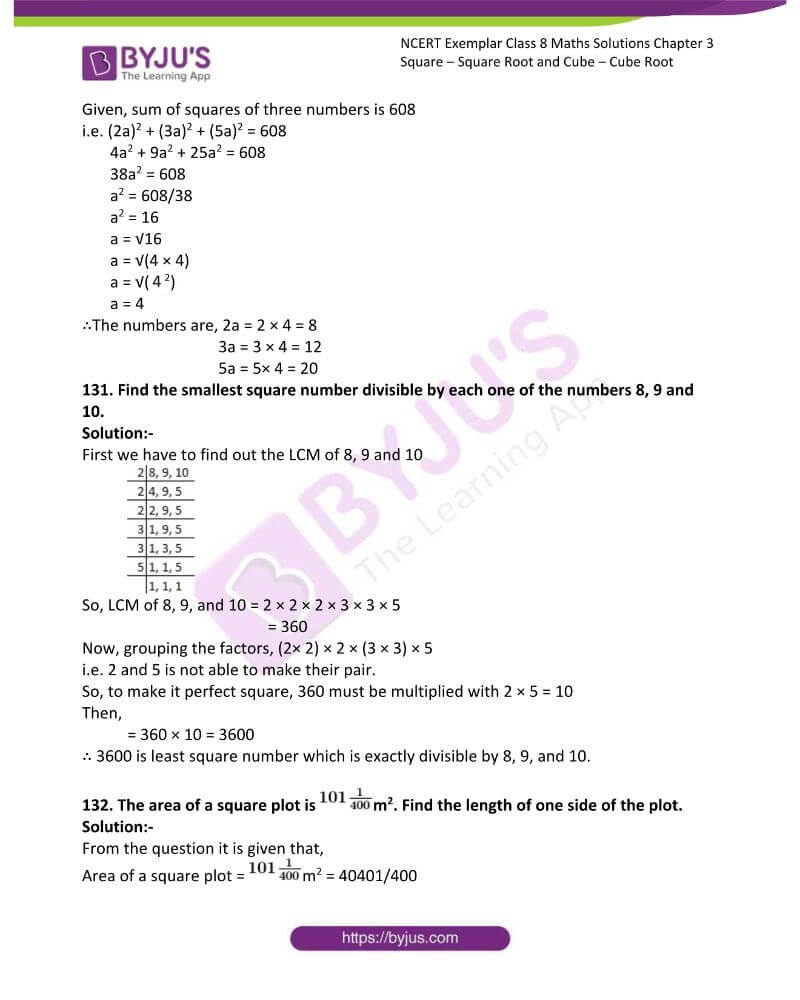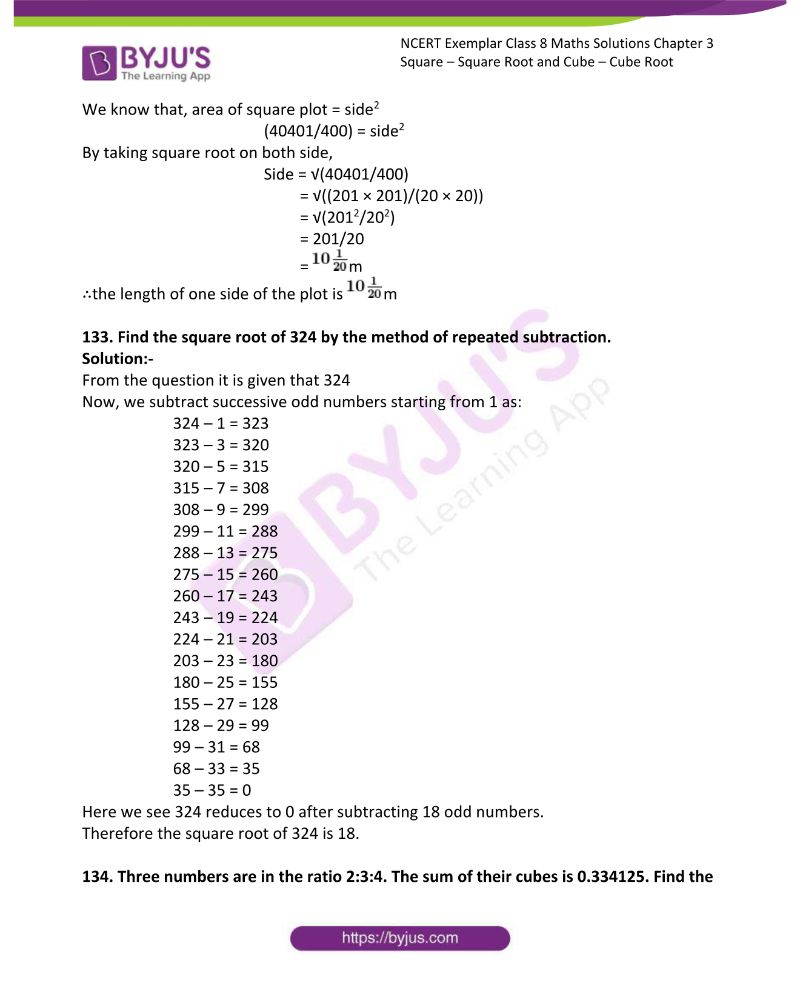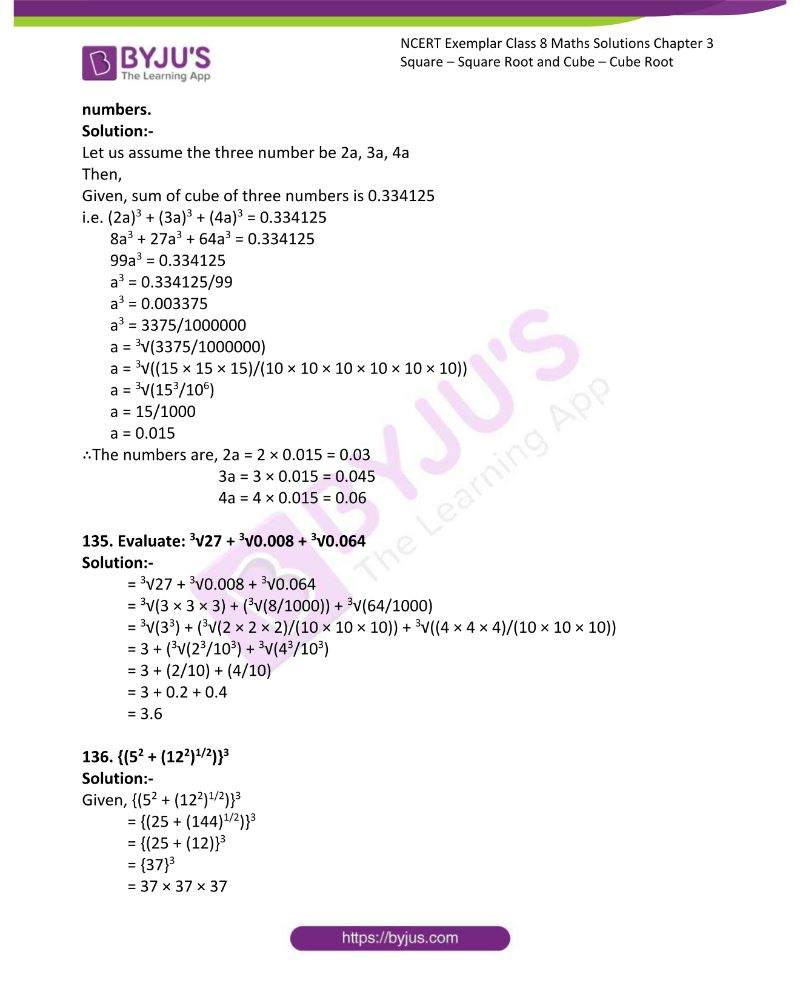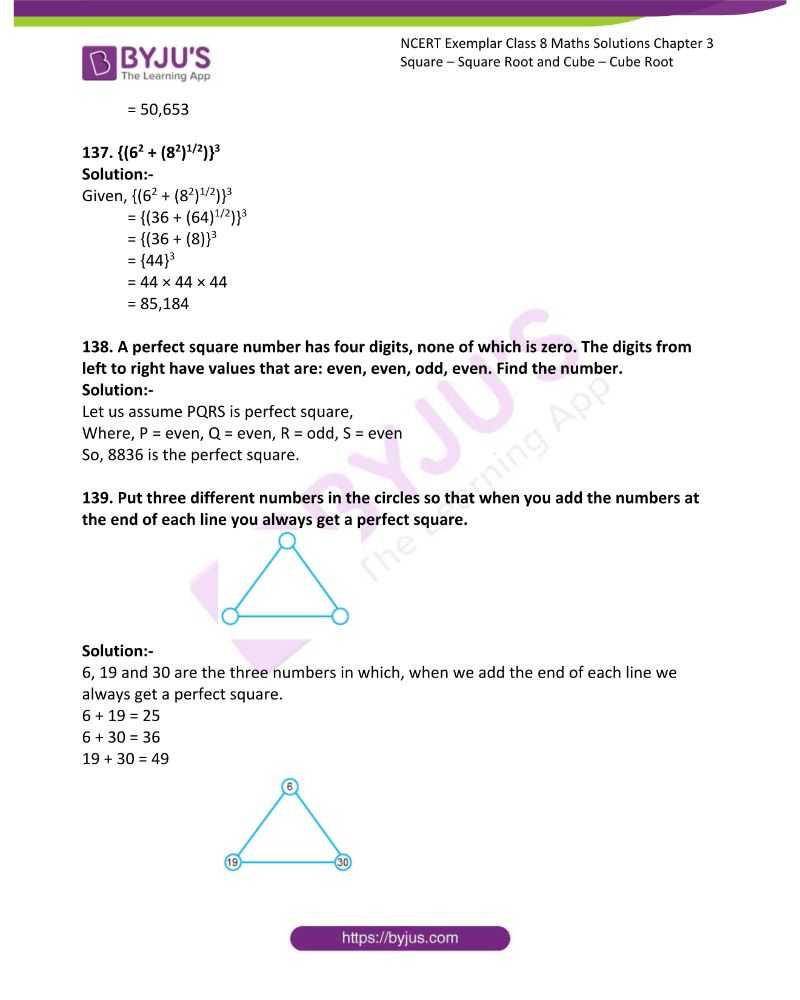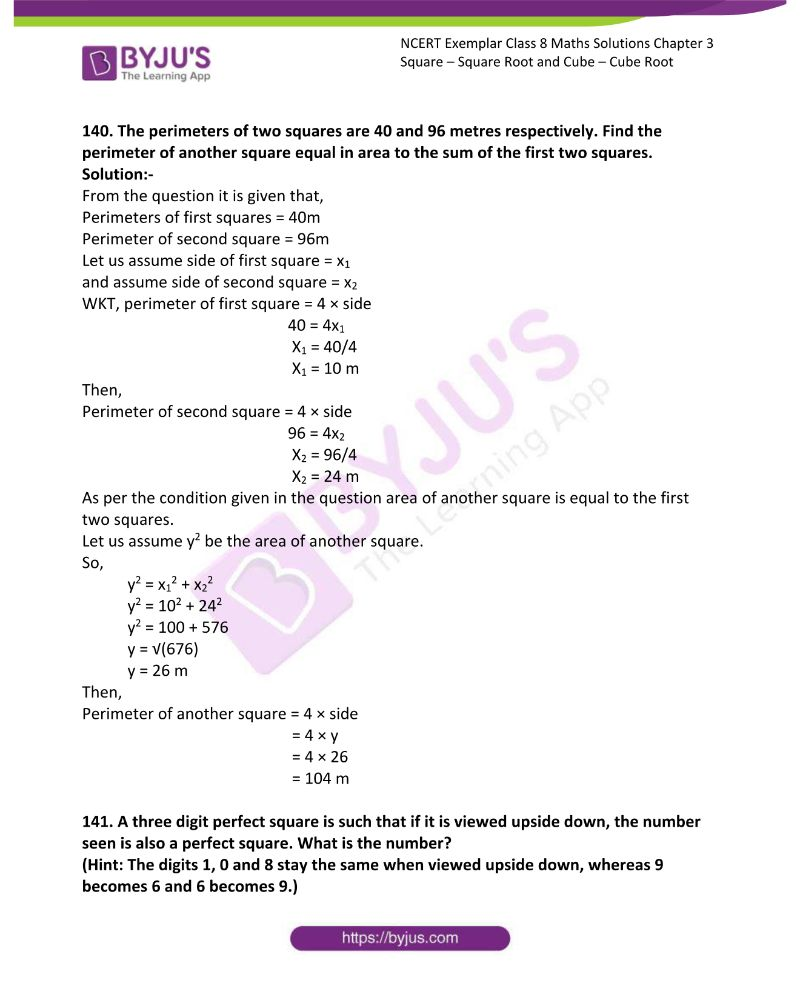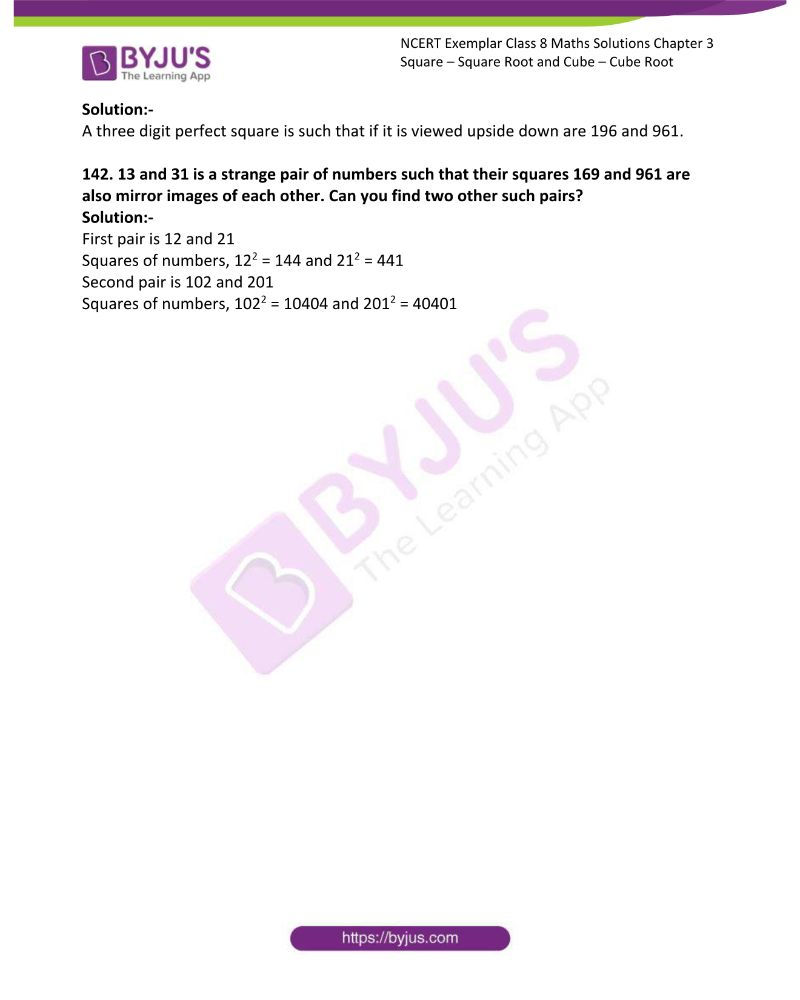### Access Answers to NCERT Exemplar Solutions Class 8 Maths Chapter 3

Exercise Page: 88

In each of the questions, 1 to 24, write the correct answer from the given four options.

1. 196 is the square of

(a) 11   (b) 12 (c) 14 (d) 16

Solution:-

(c) 14

Square of 196 is,

= 14 × 14 = 196

2. Which of the following is a square of an even number?

(a) 144 (b) 169 (c) 441 (d) 625

Solution:-

(a) 144

Square of even number is 144,

= 12 × 12 = 144

3. A number ending in 9 will have the units place of its square as

(a) 3 (b) 9 (c) 1 (d) 6

Solution:-

(c) 1

Because, the square of a number having 1 or 9 at the units place ends in 1.

Example: – 11 × 11 = 121

4. Which of the following will have 4 at the units place?

(a) 142 (b) 622 (c) 272 (d) 352

Solution:-

(b) 622

Because, the square of a number having 2 or 8 at the units place ends in 4.

62 × 62 = 3844

5. How many natural numbers lie between 52 and 62?

(a) 9 (b) 10 (c) 11 (d) 12

Solution:-

(b) 10

The natural numbers lie between 52 and 62 i.e. 25 and 36 are,

26, 27, 28, 29, 30, 31, 32, 33, 34 and 35

6. Which of the following cannot be a perfect square?

(a) 841 (b) 529 (c) 198 (d) All of the above

Solution:-

(c) 198

Because, we know that the numbers end with 2, 3, 7 and 8 are not perfect squares.

7. The one’s digit of the cube of 23 is

(a) 6 (b) 7 (c) 3 (d) 9

Solution:-

(b) 7

The one’s digit of cube 23 i.e. 23 × 23 × 23 = 12,167

So, one’s digit is 7

8. A square board has an area of 144 square units. How long is each side of the board? (a) 11 units (b) 12 units (c) 13 units (d) 14 units

Solution:-

(b) 12 units

We know that area of square = Side × Side

= 12 × 12

= 144 square units

9. Which letter best represents the location of √25 on a number line?

(a) A (b) B (c) C (d) D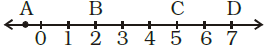Solution:-

(c) C

√25 = 5 × 5 = 52 = 25

10. If one member of a Pythagorean triplet is 2m, then the other two members are

(a) m, m2 + 1

(b) m2 + 1, m2 – 1

(c) m2, m2 – 1

(d) m2, m + 1

Solution:-

(b) m2 + 1, m2 – 1

We know that, for every natural number m > 1, 2m, m2–1 and m2 + 1 form a Pythagorean triplet.

11. The sum of successive odd numbers 1, 3, 5, 7, 9, 11, 13 and 15 is

(a) 81 (b) 64 (c) 49 (d) 36

Solution:-

(b) 64

= 1 + 3 + 5 + 7 + 9 + 11 + 13 + 15

= 64

12. The sum of first n odd natural numbers is

(a) 2n + 1 (b) n2 (c) n2 – 1 (d) n2 + 1

Solution:-

(b) n2

The sum of first n odd natural numbers is given by n2.

13. Which of the following numbers is a perfect cube?

(a) 243 (b) 216 (c) 392 (d) 8640

Solution:-

(b) 216

A perfect cube can always be expressed as the product of triplets of prime factors.

216 = 6 × 6 × 6 = 63

14. The hypotenuse of a right triangle with its legs of lengths 3x × 4x is

(a) 5x (b) 7x (c) 16x (d) 25x

Solution:-

We know that,

Pythagoras theorem = a = √(b2 + c2)

Where ‘a’ is hypotenuse and b and c are the other two legs of triangle.

Then,

a = √ ((3x)2 + (4x)2)

a = √(9x2 + 16x2)

a = √(9x2 + 16x2)

a = √(9x + 16x)

a = √25x

a = 5x

Hence the hypotenuse of the right triangle is 5x.

15. The next two numbers in the number pattern 1, 4, 9, 16, 25 … are

(a)35, 48 (b) 36, 49 (c) 36, 48 (d) 35,49

Solution:-

(b) 36, 49

The numbers given in the question are perfect square numbers.

12 = 1, 22 = 4, 32 = 9, 42 = 16, 52 = 25

So, 6 × 6 = 36 and 7 × 7 = 49

16. Which among 432, 672, 522, 592 would end with digit 1?

(a) 432 (b) 672 (c) 522 (d) 592

Solution:-

(d) 592

Because, the square of a number having 1 or 9 at the units place ends in1.

17. A perfect square can never have the following digit in its ones place.

(a) 1 (b) 8 (c) 0 (d) 6

Solution:-

(b) 8

18. Which of the following numbers is not a perfect cube?

(a) 216 (b) 567 (c) 125 (d) 343

Solution:-

(b) 567

Because, factors of 216 = 6 × 6 × 6

567 = 9 × 9 × 7

125 = 5 × 5 × 5

343 = 7 × 7 × 7

19. 3√1000 is equal to

(a) 10 (b) 100 (c) 1 (d) none of these

Solution:-

(a) 10

= 3√1000

= 3√(10 × 10 × 10)

= 3√(103)

= 10

20. If m is the square of a natural number n, then n is

(a) the square of m

(b) greater than m

(c) equal to m

(d) √m

Solution:-

(d) √m

n2 = m

Then,

= n = √m

21. A perfect square number having n digits where n is even will have square root with

(a) n + 1 digit (b) n/2 digit (c) n/3 digit (d) (n + 1)/2 digit

Solution:-

(b) n/2 digit

22. If m is the cube root of n, then n is

(a) m3 (b) √m (c) m/3 (d) 3√m

Solution:-

(a) m3

Given in the question,

3√n = m

n = m3

23. The value of √(248 + √(52 + √144)) is

(a) 14 (b) 12 (c) 16 (d) 13

Solution:-

(c) 16

√(248 + √(52 + √144))

= √(248 + √(52 + 12))

= √(248 + √(64))

= √(248 + 8)

= √(256)

= 16

24. Given that √4096 = 64, the value of √4096 + √40.96 is

(a) 74 (b) 60.4 (c) 64.4 (d) 70.4

Solution:-

(d) 70.4

√4096 + √40.96

= 64 + 6.4

= 70.4

In questions 25 to 48, fill in the blanks to make the statements true.

25. There are _________ perfect squares between 1 and 100.

Solution:-

There are 8 perfect squares between 1 and 100.

2 × 2 = 4

3 × 3 = 9

4 × 4 = 16

5 × 5 = 25

6 × 6 = 36

7 × 7 = 49

8 × 8 = 64

9 × 9 = 81

26. There are _________ perfect cubes between 1 and 1000.

Solution:-

There are 8 perfect cubes between 1 and 1000.

2 × 2 × 2 = 8

3 × 3 × 3 = 27

4 × 4 × 4 = 64

5 × 5 × 5 = 125

6 × 6 × 6 = 216

7 × 7 × 7 = 343

8 × 8 × 8 = 512

9 × 9 × 9 = 729

27. The units digit in the square of 1294 is _________.

Solution:-

The units digit in the square of 1294 is 6.

We know that, 4 or 6 at the units place ends in 6.

28. The square of 500 will have _________ zeroes.

Solution:-

The square of 500 will have 4 zeroes.

5002 = 500 × 500 = 25000

29. There are _________ natural numbers between n2 and (n + 1)2.

Solution:-

There are 2n natural numbers between n2 and (n + 1)2.

30. The square root of 24025 will have _________ digits.

Solution:-

The square root of 24025 will have 3 digits.

The number given in the question has 5 digits,

i.e. n = 5

Then,

= (n + 1)/2

= (5 + 1)/2

= 6/2

= 3

31. The square of 5.5 is _________.

Solution:-

The square of 5.5 is 30.25.

= 5.52 = 5.5 × 5.5 = 30.25

32. The square root of 5.3 × 5.3 is _________.

Solution:-

The square root of 5.3 × 5.3 is 5.3.

= √(5.3 × 5.3)

= √(28.09)

= 5.3

33. The cube of 100 will have _________ zeroes.

Solution:-

The cube of 100 will have 6 zeroes.

= 1003

= 100 × 100 × 100

= 1000000

34. 1m2 = _________ cm2.

Solution:-

We know that, 1m = 100cm

Then,

1m2 = 10000 cm2

35. 1m3 = _________ cm3.

Solution:-

We know that, 1m = 100cm

Then,

1m3 = 1000000 cm3

36. Ones digit in the cube of 38 is _________.

Solution:-

Ones digit in the cube of 38 is 2.

We know that,

38 × 38 × 38 = 54,872

37. The square of 0.7 is _________.

Solution:-

The square of 0.7 is 0.49.

= 0.72

= 0.7 × 0.7

= 0.49

38. The sum of first six odd natural numbers is _________.

Solution:-

The sum of first six odd natural numbers is 36.

The first six odd numbers are 1, 3, 5, 7, 9, and 11

Then,

Sum of first six odd numbers = 1 + 3 + 5 + 7 + 9 + 11

= 36

39. The digit at the ones place of 572 is _________.

Solution:-

The digit at the ones place of 572 is 9.

We know that, 3 or 7 at the units place ends in 9.

= 572

= 57 × 57

= 3249

40. The sides of a right triangle whose hypotenuse is 17cm are _________ and _________.

Solution:-

For every natural number m > 1, 2m, m2 –1 and m2 + 1 form a Pythagorean triplet.

Now,

m2 + 1 = (2m)2 + (m2 – 1)2

Where,

m2 + 1 = 17

m2 = 17 – 1

m2 = 16

m = √16

m = 4

Then,

2m = 2 × 4

= 8

And,

m2 – 1 = 42 – 1

= 16 -1

= 15

41. √(1.96) = _________.

Solution:-

We have,

= √(1.96)

= √(196/100)

= √((14 × 14)/(10 × 10))

= √(142 / 102)

= 14/10

= 1.4

42. (1.2)3 = _________.

Solution:-

(1.2)3 = 12/10

= (12/10) × (12/10) × (12/10)

= 1728/1000

= 1.728

43. The cube of an odd number is always an _________ number.

Solution:-

The cube of an odd number is always an odd.

44. The cube root of a number x is denoted by _________.

Solution:-

The cube root of a number x is denoted by 3√x or x1/3

45. The least number by which 125 be multiplied to make it a perfect square is _____________.

Solution:-

The least number by which 125 be multiplied to make it a perfect square is 5.

125 = 5 × 5 × 5

46. The least number by which 72 be multiplied to make it a perfect cube is _____________.

Solution:-

The least number by which 72 be multiplied to make it a perfect cube is 3.

72 = 2 × 2 × 2 × 3 × 3

47. The least number by which 72 be divided to make it a perfect cube is _____________.

Solution:-

The least number by which 72 be divided to make it a perfect cube is 9.

48. Cube of a number ending in 7 will end in the digit _______________.

Solution:-

Cube of a number ending in 7 will end in the digit 3.

In questions 49 to 86, state whether the statements are true (T) or false (F).

49. The square of 86 will have 6 at the units place.

Solution:-

True.

The square of a number having 4 or 6 at the units place ends in 6.

50. The sum of two perfect squares is a perfect square.

Solution:-

False.

Let us take two perfect square 25 and 49.

Then, sum of the two perfect square = 25 + 49 = 74

So, 74 is not perfect square.

51. The product of two perfect squares is a perfect square.

Solution:-

True.

Let us take two perfect square 4 and 9.

Then, product of two perfect square is = 4 × 9 = 36

52. There is no square number between 50 and 60.

Solution:-

True.

53. The square root of 1521 is 31.

Solution:-

False.

The square root of 1521 is 39.

54. Each prime factor appears 3 times in its cube.

Solution:-

True.

We know that, a perfect cube can always be expressed as the product of triplets of prime factors.

55. The square of 2.8 is 78.4.

Solution:-

False.

= 2.82

= (28/10)2

= 784/100

= 7.84

56. The cube of 0.4 is 0.064.

Solution:-

True.

= 0.43

= (4/10)3

= 64/1000

= 0.064

57. The square root of 0.9 is 0.3.

Solution:-

False.

= √0.9

= 0.9487

0.9 = 0.32

= 0.3 × 0.3

= 0.09

58. The square of every natural number is always greater than the number itself.

Solution:-

False.

As 1 is a natural number and the square of 1 is not greater itself rather its equal to the number itself

Thus, the given statement is not always true.

59. The cube root of 8000 is 200.

Solution:-

False

3√8000 = 20

60. There are five perfect cubes between 1 and 100.

Solution:-

False.

There are only 3 perfect cubes between 1 and 100.

2 × 2 × 2 = 8

3 × 3 × 3 = 27

4 × 4 × 4 = 64

61. There are 200 natural numbers between 1002 and 1012.

Solution:-

True.

We know that, natural numbers between a and b = b –a – 1

= 1012 – 1002 – 1

= (101 + 100) (101 – 100) – 1

= 201 – 1

= 200

62. The sum of first n odd natural numbers is n2.

Solution:-

True.

The sum of odd numbers = ∑ (2n – 1)

= (2 × n × (n + 1))/2 –n

= n2 + n – n

= n2

63. 1000 is a perfect square.

Solution:-

False.

It is not a perfect square.

1000 is a perfect cube, i.e. 10 × 10 × 10 = 1000

64. A perfect square can have 8 as its unit’s digit.

Solution:-

False.

A perfect square can never have 8 as its unit’s digit.

65. For every natural number m, (2m –1, 2m2 –2m, 2m2 –2m + 1) is a Pythagorean triplet.

Solution:-

False.

For every natural number m > 1, 2m, m2–1 and m2 + 1 form a Pythagorean triplet.

66. All numbers of a Pythagorean triplet are odd.

Solution:-

False.

Three natural numbers a, b, c are said to form a Pythagorean triplet if a2 + b2 = c2.

Then,

Pythagorean triplet as, 72 = 62 + 52

6 is not an odd number.

67. For an integer a, a3 is always greater than a2.

Solution:-

False.

Let us take one negative integer, i.e. -2

Then, -23 = -2 × -2 × -2 = -8

-22 = -2 × -2 = 4

Here, 4 > -8

Hence, -22 is greater than -23.

68. If x and y are integers such that x2 > y2, then x3 > y3.

Solution:-

False.

Let us take negative integers -2 and -3

Then,

= (-22) > (-12)

= (4) > (1)

and = -23 < -13

= -8 < -1

69. Let x and y be natural numbers. If x divides y, then x3 divides y3.

Solution:-

True.

If x divides y = y/x is a natural number

and then x3 divides y3 = y3/x3 is a natural number

70. If a2 ends in 5, then a3 ends in 25.

Solution:-

False.

Let a2 be 152

So, 152 = 225

Then, 153 = 3375

71. If a2 ends in 9, then a3 ends in 7.

Solution:-

False.

Let a2 be 72

So, 72 = 49

Then, 73 = 343

72. The square root of a perfect square of n digits will have (n + 1)/2 digits, if n is odd.

Solution:-

True.

Let au assume the perfect square has 9 digits, then its square root has 5 digits

i.e. (9 + 1)/2 = 10/2 = 5

73. Square root of a number x is denoted by √x.

Solution:-

True.

74. A number having 7 at its ones place will have 3 at the units place of its square.

Solution:-

False.

Let us take the number having 7 at its ones place i.e.47 and

Its square 472 = 2209

75. A number having 7 at its ones place will have 3 at the ones place of its cube.

Solution:-

True.

Let us take the number having 7 at its ones place i.e. 37 and

Its square 373 = 50,653

Another number is 273 = 19,683

76. The cube of a one digit number cannot be a two digit number.

Solution:-

False.

The cube of 3 .i.e. 43 = 4 × 4 × 4 = 64

77. Cube of an even number is odd.

Solution:-

False.

The cube of an even number is always an even number.

23 = 8

43 = 64

78. Cube of an odd number is even.

Solution:-

False.

The cube of odd number is always an odd number.

33 = 27

53 = 125

79. Cube of an even number is even.

Solution:-

True.

The cube of an even number is always an even number.

83 = 512

123 = 1728

80. Cube of an odd number is odd.

Solution:-

True.

The cube of odd number is always an odd number.

73 = 343

81. 999 is a perfect cube.

Solution:-

False.

82. 363 × 81 is a perfect cube.

Solution:-

False.

So, factors of 363 × 81 are 3 × 11 × 11 × 3 × 3 × 3 × 3

= 33 × 11 × 11 × 3 × 3

83. Cube roots of 8 are +2 and –2.

Solution:-

False.

Because, cube root of 8 is 2 only.

i.e. 2 × 2 × 2 = 8

84. 3√ (8 + 27) = 3√ (8) + 3√ (27).

Solution:-

False.

Let us consider LHS = 3√ (8 + 27)

= 3√ (35)

Then, RHS = 3√8 + 3√27

= 2 + 3

= 5

By comparing LHS and RHS

LHS ≠ RHS

3√ (35) ≠ 5

85. There is no cube root of a negative integer.

Solution:-

False.

Let us take -27

Cube root of -27 i.e. 3√-27 = 3√((-3) × (-3) × (-3))

= -3

86. Square of a number is positive, so the cube of that number will also be positive.

Solution:-

False.

Let us take -3

Now, square of the -3 i.e. -32 = 9

Then, cube of the same number i.e. -33 = -27

Solve the following questions.

87. Write the first five square numbers.

Solution:-

The first five square numbers are,

1 × 1 = 1

2 × 2 = 4

3 × 3 = 9

4 × 4 = 16

5 × 5 = 25

88. Write cubes of first three multiples of 3.

Solution:-

The first three multiple of 3 are 3, 6 and 9

Then,

Cubes of first three multiples of 3,

= 33 = 3 × 3 × 3 = 27

= 63 = 6 × 6 × 6 = 216

= 93 = 9 × 9 × 9 = 729

89. Show that 500 is not a perfect square.

Solution:-

Factors of 500 are 2 × 2 × 5 × 5 × 5

Now,

Group the factors into pairs,

500 = (2 × 2) × (5 × 5 × 5)

= 22 × 53

So, the prime factors 2 and 5 do not occur in pairs.

Therefore, 500 is not a perfect square.

90. Express 81 as the sum of first nine consecutive odd numbers.

Solution:-

The first nine consecutive odd numbers are 1, 3, 5, 7, 9, 11, 13, 15 and 17

Then,

We know that 81 is a perfect square,

81 = (9)2

Now,

The sum of first nine consecutive odd numbers,

= 1 + 3 + 5 + 7 + 9 + 11 + 13 + 15 + 17

= 81

91. Using prime factorisation, find which of the following are perfect squares.

(a) 484

Solution:-

First we have to find out the factors by using prime factorisation method.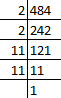So, prime factors of 484 = 2 × 2 × 11 × 11

Now, grouping the prime factors = (2 × 2) × (11 × 11)

= 22 × 112

There is no unpaired factor remains here.

∴484 is a perfect square.

(b) 11250

Solution:-

First we have to find out the factors by using prime factorisation method.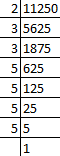So, prime factors of 11250 = 2 × 3 × 3 × 5 × 5 × 5 × 5

Now, grouping the prime factors = 2 × (3 × 3) × (5 × 5) × (5 × 5)

= 2 × 32 × 52 × 52

Factor 2 has no pair.

∴11250 is not a perfect square.

(c) 841

Solution:-

First we have to find out the factors by using prime factorisation method.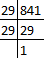So, prime factors of 841 = 29 × 29

Now, grouping the prime factors = (29 × 29)

= 292

There is no unpaired factor remains here.

∴841 is a perfect square.

(d) 729

Solution:-

First we have to find out the factors by using prime factorisation method.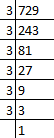So, prime factors of 729 = 3 × 3 × 3 × 3 × 3 × 3

Now, grouping the prime factors = (3 × 3) × (3 × 3) × (3 × 3)

= 32 × 32 × 32

There is no unpaired factor remains here.

∴729 is a perfect square.

92. Using prime factorisation, find which of the following are perfect cubes.

(a)128

Solution:-

First we have to find out the factors by using prime factorisation method.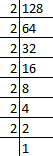So, prime factors of 128 = 2 × 2 × 2 × 2 × 2 × 2 × 2

Now, grouping the prime factors = (2 × 2 × 2) × (2 × 2 × 2) × 2

= 23 × 23 × 2

Factor 2 remains unpaired after grouping in triplets.

∴128 is not a perfect cube.

(b) 343

Solution:-

First we have to find out the factors by using prime factorisation method.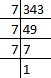So, prime factors of 343 = 7 × 7 × 7

Now, grouping the prime factors = (7 × 7 × 7)

= 73

There is no unpaired factor remains here.

∴343 is a perfect cube.

(c) 729

Solution:-

First we have to find out the factors by using prime factorisation method.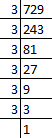So, prime factors of 729 = 3 × 3 × 3 × 3 × 3 × 3

Now, grouping the prime factors = (3 × 3 × 3) × (3 × 3 × 3)

= 33 × 33

There is no unpaired factor remains here.

∴729 is a perfect cube.

(d) 1331

Solution:-

First we have to find out the factors by using prime factorisation method.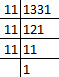So, prime factors of 1331 = 11 × 11 × 11

Now, grouping the prime factors = (11 × 11 × 11)

= 113

There is no unpaired factor remains here.

∴1331 is a perfect cube.

93. Using distributive law, find the squares of

(a)101

Solution:-

By using distributive law,

We have,

101 = 100 + 1

So,

1012 = (100 + 1)2

= (100 + 1) (100 + 1)

= 100 (100 + 1) + 1 (100 + 1)

= ((100 × 100) + (100 × 1)) + ((1 × 100) + (1 × 1))

= 10000 + 100 + 100 + 1

= 10201

∴The square of the given number i.e. 1012 = 10201

(b) 72

Solution:-

By using distributive law,

We have,

72 = 70 + 2

So,

722 = (70 + 2)2

= (70 + 2) (70 + 2)

= 70 (70 + 2) + 2 (70 + 2)

= ((70 × 70) + (70 × 2)) + ((2 × 70) + (2 × 2))

= 4900 + 140 + 140 + 4

= 5184

∴The square of the given number i.e. 722 = 5184

94. Can a right triangle with sides 6cm, 10cm and 8cm be formed? Give reason.

Solution:-

From the question it is given that,

Sides of the right angle triangle are 6 cm, 10 cm and 8 cm

We know that, the square one side must be equal to the sum of square of other two sides of right angle triangle.

i.e. a2 = b2 + c2

Where, a = 10 cm, b = 8 cm, c = 6 cm

102 = 82 + 62

100 = 64 + 36

100 = 100

∴Right angle triangle is formed by the given sides 6cm, 10cm and 8cm.

95. Write the Pythagorean triplet whose one of the numbers is 4.

Solution:-

Given, one of the number in the Pythagorean triplet i.e. 4.

Then,

2m = 4

m = 4/2

m = 2

So,

= m2 + 1

= 22 + 1

= 4 + 1

= 5

And,

= m2 – 1

= 22 – 1

= 4 – 1

= 3

∴The Pythagorean triplet is 3, 4 and 5.

96. Using prime factorisation, find the square roots of

(a)11025

Solution:-

First we have to find out the factors by using prime factorisation method.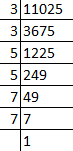So, prime factors of 11025 = 3 × 3 × 5 × 5 × 7 × 7

Now, grouping the prime factors = (3 × 3) × (5 × 5) × (7 × 7)

Then, Square root of 11025 = √11025

= √((3 × 3) × (5 × 5) × (7 × 7))

= √(32 × 52 × 72)

= 3 × 5 × 7

= 105

∴The Square root of 11025 is 105.

(b) 4761

Solution:-

First we have to find out the factors by using prime factorisation method.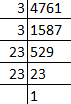So, prime factors of 4761 = 3 × 3 × 23 × 23

Now, grouping the prime factors = (3 × 3) × (23 × 23)

Then, Square root of 4761 = √4761

= √((3 × 3) × (23 × 23))

= √(32 × 232)

= 3 × 23

= 69

∴The Square root of 4761 is 69.

97. Using prime factorisation, find the cube roots of

(a)512

Solution:-

First we have to find out the factors by using prime factorisation method.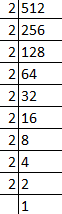So, prime factors of 512 = 2 × 2 × 2 × 2 × 2 × 2 × 2 × 2 × 2

Now, grouping the prime factors = (2 × 2 × 2) × (2 × 2 × 2) × (2 × 2 × 2)

Then, cube root of 512 = 3√512

= 3√((2 × 2 × 2) × (2 × 2 × 2) × (2 × 2 × 2))

= 3√(23 × 23 × 23)

= 2 × 2 × 2

= 8

∴The cube root of 512 is 8.

(b) 2197

Solution:-

First we have to find out the factors by using prime factorisation method.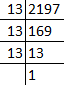So, prime factors of 2197 = 13 × 13 × 13

Now, grouping the prime factors = (13 × 13 × 13)

Then, cube root of 2197 = 3√2197

= 3√(13 × 13 × 13)

= 3√(133)

= 13

∴The cube root of 2197 is 13.

98. Is 176 a perfect square? If not, find the smallest number by which it should be multiplied to get a perfect square.

Solution:-

First we have to find out the factors by using prime factorisation method.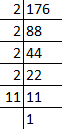So, prime factors of 176 = 2 × 2 × 2 × 2 × 11

Now, grouping the prime factors = (2 × 2) × (2 × 2) × (11)

= 22 × 22 × 11

Factor 11 has no pair.

∴176 is not a perfect square.

The smallest number it should be multiplied to get a perfect square is 11.

Then,

176 × 11 = 1936

Factors of 1936 = 2 × 2 × 2 × 2 × 11 × 11

= √1936

= √(22 × 22 × 112)

= 2 × 2 × 11

= 44

∴Square root of 1936 is 44.

99. Is 9720 a perfect cube? If not, find the smallest number by which it should be divided to get a perfect cube.

Solution:-

First we have to find out the factors by using prime factorisation method.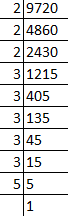So, prime factors of 9720 = 2 × 2 × 2 × 3 × 3 × 3 × 3 × 3 × 5

Now, grouping the prime factors = (2 × 2 × 2) × (3 × 3 × 3) × 3 × 3 × 5

= 23 × 33 × 3 × 3 × 5

Factors 3 and 4 has no pair.

∴9720 is not a perfect cube.

The smallest number it should be divided to get a perfect cube is 3 × 3 × 5 = 45.

Then,

= 9720 ÷ 45

= 216

Factors of 216 = (2 × 2 × 2) × (3 × 3 × 3)

3√216 = 3√((2 × 2 × 2) × (3 × 3 × 3))

= 3√(23 × 32)

= 6

100. Write two Pythagorean triplets each having one of the numbers as 5.

Solution:-

Given, one of the number in the Pythagorean triplet i.e. 5.

= m2 + 1 = 5

= m2 = 4

= m = 2

Then,

2m = 2 × 2

m = 4

So,

And,

= m2 – 1

= 22 – 1

= 4 – 1

= 3

∴The Pythagorean triplet is 3, 4 and 5.

Similarly,

The other Pythagorean triplet is 5, 12 and 13.

101. By what smallest number should 216 be divided so that the quotient is a perfect square. Also find the square root of the quotient.

Solution:-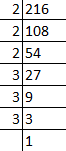Factors of 216 = 2 × 2 × 2 × 3 × 3 × 3

Now, grouping the prime factors = (2 × 2) × 2 × (3 × 3) × 3

Factors 2 and 3 has no pair.

∴216 is not a perfect square.

The smallest number it should be divided to get a perfect square is 3 × 2 = 6.

Then,

= 216 ÷ 6

= 36

Factors of 36 = 6 × 6

√36 = √(6× 6)

= √(6)

= 6

∴36 is a perfect square and 6 is the square root of 36.

102. By what smallest number should 3600 be multiplied so that the quotient is a perfect cube. Also find the cube root of the quotient.

Solution:-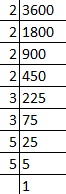Factors of 3600 = 2 × 2 × 2 × 2 × 3 × 3 × 5 × 5

Now, grouping the prime factors = (2 × 2 × 2) × 2 × 3 × 3 × 5 × 5

Factors 2, 3 and 5 has no pair.

∴3600 is not a perfect cube.

The smallest number it should be multiplied to get a perfect cube is 2 × 2 × 3 × 5 = 60

Then,

Product = 3600 × 60 = 216000

Factors of 21600 = 2 × 2 × 2 × 2 × 2 × 2 × 3 × 3 × 3 × 5 × 5 × 5

= 3√216000

= 3√(23 × 23 × 33 × 53)

= 2 × 2 × 3 × 5

= 60

103. Find the square root of the following by long division method.

(a)1369

Solution:-

We have,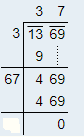∴ √1369 = 37

(b) 5625

Solution:-

We have,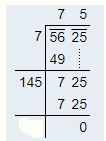∴ √5625 = 75

104. Find the square root of the following by long division method.

(a)27.04

Solution:-

We have,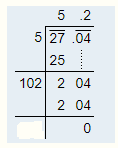∴ √27.04 = 5.2

(b) 1.44

Solution:-

We have,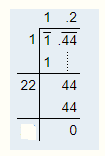∴ √1.44 = 1.2

105. What is the least number that should be subtracted from 1385 to get a perfect square? Also find the square root of the perfect square.

Solution:-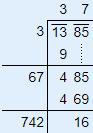From the long division method, the least number that should be subtracted from 1385 to get a perfect square is 16.

Now,

= 1385 – 16

= 1369

So,

√1369 = √(37 × 37)

= √372

= 37

∴The square root of a perfect square number 1369 is 37.

106. What is the least number that should be added to 6200 to make it a perfect square?

Solution:-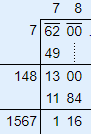So, 782 = 6084

By comparing 6084 and 6200, 6084 < 6200

Then, next perfect square is 792 = 6241

∴The least number is (6241 – 6200) = 41, which should be added to 6200 to get a perfect square.

The required perfect square number is 6241

So,

√6241 = √(41 × 41)

= √412

= 41

107. Find the least number of four digits that is a perfect square.

Solution:-

We know that, the least number of four digit is 1000

By using long division method we can able to find a perfect square,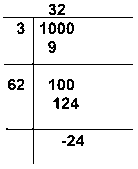∴The least number of four digits that is a perfect square = 1000 + 24

= 1024

108. Find the greatest number of three digits that is a perfect square.

Solution:-

Solution:-

We know that, the greatest number of three digit is 999.

By using long division method we can able to find a perfect square,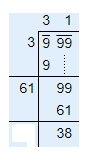∴The greatest number of three digits that is a perfect square = 999 – 38

= 961

109. Find the least square number which is exactly divisible by 3, 4, 5, 6 and 8.

Solution:-

First we have to find out the LCM of 3, 4, 5, 6 and 8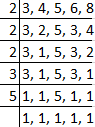So, LCM of 3, 4, 5, 6 and 8 = 2 × 2 × 2 × 3 × 5

= 120

Now, grouping the factors, (2× 2) × 2× 3× 5

i.e. 2,3 and 5 is not able to make their pair.

So, to make it perfect square, 120 must be multiplied with 2× 3× 5 = 30

Then,

= 120× 30 = 3600

∴ 3600 is least square number which is exactly divisible by 3, 4, 5, 6, and 8.

110. Find the length of the side of a square if the length of its diagonal is 10cm.

Solution:-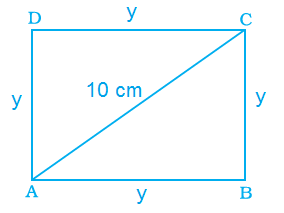From the question it is given that,

Length of Diagonal = 10 cm

Let us assume square ABCD.

Sides AB = y, BC = y, CD = y, DA = y

Diagonal AC = 10 cm

Now, consider triangle ABC

By the rule of Pythagoras theorem,

AC2 = AB2 + BC2

102 = y2 + y2

100 = 2y2

y2 = 100/2

y2 = 50

y = √50 cm

y = 5√2 cm

∴The length of the side of square is √50 cm or 5√2.

111. A decimal number is multiplied by itself. If the product is 51.84, find the number.

Solution:-

Let us assume decimal number be ‘a’.

We know that, a × a = a2

So,

a × a = 51.84

a2 = 51.84

a = √51.84

The required number is found out by using long division method.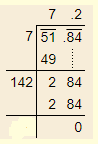Then,

a = √51.84

a = 7.2

∴7.2 is the decimal number is multiplied by itself and its product is 51.84.

112. Find the decimal fraction which when multiplied by itself gives 84.64.

Solution:-

Let us assume decimal fraction be ‘a’.

We know that, a × a = a2

So,

a × a = 84.64

a2 = 84.64

a = √84.64

The required number is found out by using long division method.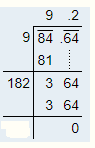Then,

a = √84.64

a = 9.2

∴9.2 is the decimal fraction is multiplied by itself and its product is 84.64.

113. A farmer wants to plough his square field of side 150m. How much area will he have to plough?

Solution:-

From the question it is given that,

Length of the side of square field = 150 m

We know that, are of square = side × side

So,

Area of the square field = 150 × 150

= 22,500 m2

∴The farmer have to plough 22,500 m2 area.

114. What will be the number of unit squares on each side of a square graph paper if the total number of unit squares is 256?

Solution:-

From the question it is given that,

The total number of unit squares is = 256.

Let us assume the number be ‘a’

We know that, a × a = a2

So,

a × a = 256

a2 = 256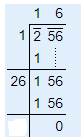a = √256

a = √(16 × 16)

a = √162

a = 16

∴The number of unit squares are 16.

115. If one side of a cube is 15m in length, find its volume.

Solution:-

From the question it is given that,

Length of one side of the a cube = 15 m

We know that, volume of cube = (side)3

= 153

= 15 × 15 × 15

= 3375 m3

∴The volume of cube is 3375 m3.

116. The dimensions of a rectangular field are 80m and 18m. Find the length of its diagonal.

Solution:-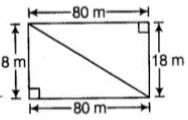From the question it is given that,

Length of rectangular field = 80 m

Breadth of rectangular field = 18 m

Then,

Length of diagonal = √(length2 + breadth2)

= √(802 + 182)

= √(6400 + 324)

= √6724

= 82 m

∴The Length of diagonal is 82 m.

117. Find the area of a square field if its perimeter is 96m.

Solution:-

Given, perimeter of the square field = 96 m

We know that, perimeter of square = 4 × side

Then,

96 = 4 × side

Side = 96/4

Side = 24 m

So, the length of the side of square = 24 m

Now,

Area of the square field = (side)2

= 242

= 576 m2

∴The area of the square field is 576m2.

118. Find the length of each side of a cube if its volume is 512 cm3.

Solution:-

From the question it is given that,

Volume of the cube = 512 cm3

We know that,

Volume of cube = side3

512 = side3

By taking cube root on both the side,

3√512 = side

Side = 3√(8 × 8 × 8)

Side = 3√ (8)3

Side = 8 cm

∴The length of each side of a cube is 8 cm.

119. Three numbers are in the ratio 1:2:3 and the sum of their cubes is 4500. Find the numbers.

Solution:-

Let us assume the three number be a, 2a, 3a

Then,

Given, sum of cube of three numbers is 4500

i.e. a3 + (2a)3 + (3a)3 = 4500

a3 + 8a3 + 27a3 = 4500

36a3 = 4500

a3 = 4500/36

a3 = 125

a = 3√125

a = 3√(5 × 5 × 5)

a = 3√(53)

a = 5

∴The numbers are, a = 5

2a = 2 × 5 = 10

3a = 3 × 5 = 15

120. How many square metres of carpet will be required for a square room of side 6.5m to be carpeted.

Solution:-

Given, side of square room = 6.5 m

So, area of square room = 6.52

= 6.5 × 6.5

= 42.25 m2

121. Find the side of a square whose area is equal to the area of a rectangle with sides 6.4m and 2.5m.

Solution:-

From the question it is given that,

Length of rectangle = 6.4 m

Breadth of rectangle = 2.5 m

Area of rectangle = length × breadth

= 6.4 × 2.5

= 16 m2

And also it’s given in the question i.e. area of square is equal to the area of rectangle.

Let us assume area of square be ‘a’.

Area of square = Area of rectangle

a × a = 16

a2 = 16

By taking square root on both side

a = √16

a = √(4 × 4)

a = √42

a = 4m2

We know that, area of square = side2

4 = side2

By taking square root on both side

side = √4

side = √(2 × 2)

side = √22

side = 2m

122. Difference of two perfect cubes is 189. If the cube root of the smaller of the two numbers is 3, find the cube root of the larger number.

Solution:-

From the question it is given that,

Difference of two perfect cubes = 189

The cube root of the smaller of the two numbers = 3

So, cube of smaller number = 33

= 3 × 3 × 3

= 27

Let us assume the cube root of larger number be a3

Then,

As per the condition given in the question,

a3 – 27 = 189

a3 = 189 + 27

a3 =216

By taking cube root on both side,

a = 3√216

a = 3√(6 × 6 × 6)

a = 3√(63)

a = 6

∴The cube root of the larger number is 6.

123. Find the number of plants in each row if 1024 plants are arranged so that number of plants in a row is the same as the number of rows.

Solution:-

From the question it is given that total number of plants arranged = 1024

Let us assume number of plants in each row be ‘a’

As per the condition given in the question,

Number of plants in a row is the same as the number of rows i.e. ‘a’

Then,

Total number of plants = a × a

1024 = a2

By taking square root on both side,

a = √(1024)

a = √(32 × 32)

a = √(32)2

a = 32

∴The number of plants in each row is 32.

124. A hall has a capacity of 2704 seats. If the number of rows is equal to the number of seats in each row, then find the number of seats in each row.

Solution:-

From the question it is given that total number of seats = 2704

Let us assume number of seats in each row be ‘a’

As per the condition given in the question,

Number of rows is equal to the number of seats in each row i.e. ‘a’

Then,

Total number of seats = a × a

2704 = a2

By taking square root on both side,

a = √(2704)

a = √(52 × 52)

a = √(52)2

a = 52

∴The number of seats in each row is 52.

125. A General wishes to draw up his 7500 soldiers in the form of a square. After arranging, he found out that some of them are left out. How many soldiers were left out?

Solution:-

From the question it is given that total number of soldiers = 7500

By using long division method we can able to find number of soldiers left out,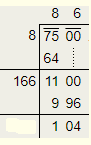∴104 soldiers were left out.

126. 8649 students were sitting in a lecture room in such a manner that there were as many students in the row as there were rows in the lecture room. How many students were there in each row of the lecture room?

Solution:-

From the question it is given that,

Total number of students were sitting in a lecture room = 8649

Let us assume number of students in each row be ‘a’

Then,

Total number of students = a × a

8649 = a2

By taking square root on both side,

a = √(8649)

a = √(93 × 93)

a = √(93)2

a = 93

∴The number of students in each row is 93.

127. Rahul walks 12m north from his house and turns west to walk 35m to reach his friend’s house. While returning, he walks diagonally from his friend’s house to reach back to his house. What distance did he walk while returning?

Solution:-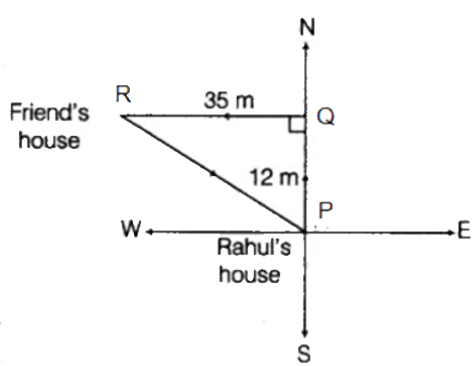From above the figure,

Consider the triangle PQR.

We know that, Pythagoras theorem,

PR2 = PQ2 + QR2

PR2 = 122 + 352

PR2 = 144 + 1225

PR2 = 1369

PR = √1369

PR = 37m

∴Rahul walked 37m distance for returning to his house.

128. A 5.5m long ladder is leaned against a wall. The ladder reaches the wall to a height of 4.4m. Find the distance between the wall and the foot of the ladder.

Solution:-

Let us consider the right angle triangle PQR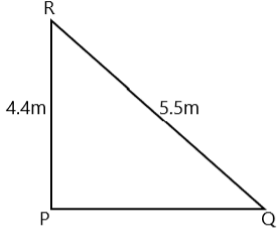Given,

Long ladder i.e. RQ = 5.5 m

The ladder reaches the wall to a height i.e. PR = 4.4 m

From Pythagoras theorem,

RQ2 = RP2 + PQ2

5.52 = 4.42 + PQ2

PQ2 = 5.52 – 4.42

PQ = √(5.52 – 4.42)

PQ = √(30.25 – 19.36)

PQ = √10.89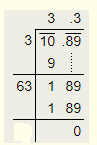PQ = 3.3

∴The distance between the wall and the foot of the ladder is 3.3 m.

129. A king wanted to reward his advisor, a wise man of the kingdom. So he asked the wiseman to name his own reward. The wiseman thanked the king but said that he would ask only for some gold coins each day for a month. The coins were to be counted out in a pattern of one coin for the first day, 3 coins for the second day, 5 coins for the third day and so on for 30 days. Without making calculations, find how many coins will the advisor get in that month?

Solution:-

From the question it is given that,

Total coins the advisor get at the end = 1 + 3 + 5 +…..

The coins order is in the series of odd natural number,

So, the number of terms (n) = 30

Then,

Sum of odd natural numbers = n2

= 302

= 900

∴ Total coins the advisor get at the end is 900.

130. Find three numbers in the ratio 2:3:5, the sum of whose squares is 608.

Solution:-

Let us assume the three number be 2a, 3a, 5a

Then,

Given, sum of squares of three numbers is 608

i.e. (2a)2 + (3a)2 + (5a)2 = 608

4a2 + 9a2 + 25a2 = 608

38a2 = 608

a2 = 608/38

a2 = 16

a = √16

a = √(4 × 4)

a = √(42)

a = 4

∴The numbers are, 2a = 2 × 4 = 8

3a = 3 × 4 = 12

5a = 5× 4 = 20

131. Find the smallest square number divisible by each one of the numbers 8, 9 and 10.

Solution:-

First we have to find out the LCM of 8, 9 and 10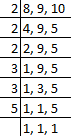So, LCM of 8, 9, and 10 = 2 × 2 × 2 × 3 × 3 × 5

= 360

Now, grouping the factors, (2× 2) × 2 × (3 × 3) × 5

i.e. 2 and 5 is not able to make their pair.

So, to make it perfect square, 360 must be multiplied with 2 × 5 = 10

Then,

= 360 × 10 = 3600

∴ 3600 is least square number which is exactly divisible by 8, 9, and 10.

132. The area of a square plot is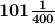m2. Find the length of one side of the plot.

Solution:-

From the question it is given that,

Area of a square plot =m2 = 40401/400

We know that, area of square plot = side2

(40401/400) = side2

By taking square root on both side,

Side = √(40401/400)

= √((201 × 201)/(20 × 20))

= √(2012/202)

= 201/20

=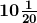m

∴the length of one side of the plot ism

133. Find the square root of 324 by the method of repeated subtraction.

Solution:-

From the question it is given that 324

Now, we subtract successive odd numbers starting from 1 as:

324 – 1 = 323

323 – 3 = 320

320 – 5 = 315

315 – 7 = 308

308 – 9 = 299

299 – 11 = 288

288 – 13 = 275

275 – 15 = 260

260 – 17 = 243

243 – 19 = 224

224 – 21 = 203

203 – 23 = 180

180 – 25 = 155

155 – 27 = 128

128 – 29 = 99

99 – 31 = 68

68 – 33 = 35

35 – 35 = 0

Here we see 324 reduces to 0 after subtracting 18 odd numbers.

Therefore the square root of 324 is 18.

134. Three numbers are in the ratio 2:3:4. The sum of their cubes is 0.334125. Find the numbers.

Solution:-

Let us assume the three number be 2a, 3a, 4a

Then,

Given, sum of cube of three numbers is 0.334125

i.e. (2a)3 + (3a)3 + (4a)3 = 0.334125

8a3 + 27a3 + 64a3 = 0.334125

99a3 = 0.334125

a3 = 0.334125/99

a3 = 0.003375

a3 = 3375/1000000

a = 3√(3375/1000000)

a = 3√((15 × 15 × 15)/(10 × 10 × 10 × 10 × 10 × 10))

a = 3√(153/106)

a = 15/1000

a = 0.015

∴The numbers are, 2a = 2 × 0.015 = 0.03

3a = 3 × 0.015 = 0.045

4a = 4 × 0.015 = 0.06

135. Evaluate: 3√27 + 3√0.008 + 3√0.064

Solution:-

= 3√27 + 3√0.008 + 3√0.064

= 3√(3 × 3 × 3) + (3√(8/1000)) + 3√(64/1000)

= 3√(33) + (3√(2 × 2 × 2)/(10 × 10 × 10)) + 3√((4 × 4 × 4)/(10 × 10 × 10))

= 3 + (3√(23/103) + 3√(43/103)

= 3 + (2/10) + (4/10)

= 3 + 0.2 + 0.4

= 3.6

136. {(52 + (122)1/2)}3

Solution:-

Given, {(52 + (122)1/2)}3

= {(25 + (144)1/2)}3

= {(25 + (12)}3

= {37}3

= 37 × 37 × 37

= 50,653

137. {(62 + (82)1/2)}3

Solution:-

Given, {(62 + (82)1/2)}3

= {(36 + (64)1/2)}3

= {(36 + (8)}3

= {44}3

= 44 × 44 × 44

= 85,184

138. A perfect square number has four digis, none of which is zero. The digits from left to right have values that are: even, even, odd, even. Find the number.

Solution:-

Let us assume PQRS is perfect square,

Where, P = even, Q = even, R = odd, S = even

So, 8836 is the perfect square.

139. Put three different numbers in the circles so that when you add the numbers at the end of each line you always get a perfect square.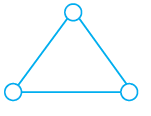Solution:-

6, 19 and 30 are the three numbers in which, when we add the end of each line we always get a perfect square.

6 + 19 = 25

6 + 30 = 36

19 + 30 = 49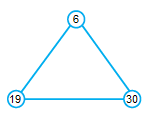140. The perimeters of two squares are 40 and 96 metres respectively. Find the perimeter of another square equal in area to the sum of the first two squares.

Solution:-

From the question it is given that,

Perimeters of first squares = 40m

Perimeter of second square = 96m

Let us assume side of first square = x1

and assume side of second square = x2

WKT, perimeter of first square = 4 × side

40 = 4x1

X1 = 40/4

X1 = 10 m

Then,

Perimeter of second square = 4 × side

96 = 4x2

X2 = 96/4

X2 = 24 m

As per the condition given in the question area of another square is equal to the first two squares.

Let us assume y2 be the area of another square.

So,

y2 = x12 + x22

y2 = 102 + 242

y2 = 100 + 576

y = √(676)

y = 26 m

Then,

Perimeter of another square = 4 × side

= 4 × y

= 4 × 26

= 104 m

141. A three digit perfect square is such that if it is viewed upside down, the number seen is also a perfect square. What is the number?

(Hint: The digits 1, 0 and 8 stay the same when viewed upside down, whereas 9 becomes 6 and 6 becomes 9.)

Solution:-

A three digit perfect square is such that if it is viewed upside down are 196 and 961.

142. 13 and 31 is a strange pair of numbers such that their squares 169 and 961 are also mirror images of each other. Can you find two other such pairs?

Solution:-

First pair is 12 and 21

Squares of numbers, 122 = 144 and 212 = 441

Second pair is 102 and 201

Squares of numbers, 1022 = 10404 and 2012 = 40401

Download BYJU’S-The Learning App and get personalized videos, explaining the concepts of squares, square roots, cube and cube roots, etc. and experience a new way of learning to understand the concepts easily.

## Frequently Asked Questions on NCERT Exemplar Solutions for Class 8 Maths Chapter 3

### Write the key benefits of NCERT Exemplar Solutions for Class 8 Maths Chapter 3.

1. The solutions provided in NCERT Exemplar Solutions for Class 8 Maths Chapter 3 are offered in a step by step approach for a comfortable and better understanding of concepts.
2. It also provides explanatory diagrams and tables for comparative study, which creates an interest in learning.
3. These solutions facilitate the students to build a good knowledge of basic as well as advanced mathematical concepts.
4. It also helps students in retaining and quickly retrieving the concepts.

### How NCERT Exemplar Solutions for Class 8 Maths Chapter 3 helpful for Class 9 students?

The NCERT Exemplar Solutions for Class 8 Maths Chapter 3 can help the students to clear their doubts and prepare themselves for the exam in a better way. The NCERT questions not only help for board exam preparation but also for different competitive exams. Students are advised to follow the NCERT Exemplar Solutions for Class 8 Chapter 3 on a regular basis to get in-depth knowledge about all the concepts covered in the syllabus. These solutions are prepared by our BYJU’S expert team, focusing completely on accuracy. Using these solutions regularly makes you score high marks in board exams.

### What are the concepts covered in NCERT Exemplar Solutions for Class 8 Maths Chapter 3?

The concepts covered in NCERT Exemplar Solutions for Class 8 Maths Chapter 3 are
1. Squares and square roots
2. Properties of square numbers
3. Find the squares of a number
4. Pythagorean triplets
5. Finding the square root of a number in general form by prime factorization and by division method
6. Cubes and their prime factors
7. Finding a perfect cube
8. Finding cube roots by prime factorization method and estimation method

Also, Check